# Tenth maths guide for polynomials, exercise 3.1, 3.2, 3.3, 3.4.

Tenth class mathematics solutions for text book problems. These are very easy to understand.

Maths solutions for 10th class Andhra pradesh and Telangana.

SSC maths solutions for chapter 3 polynomials.

You can also see the solutions for

2. Sets

# Solutions for class 10 maths chapter 3

## Polynomials

Exercise 3.1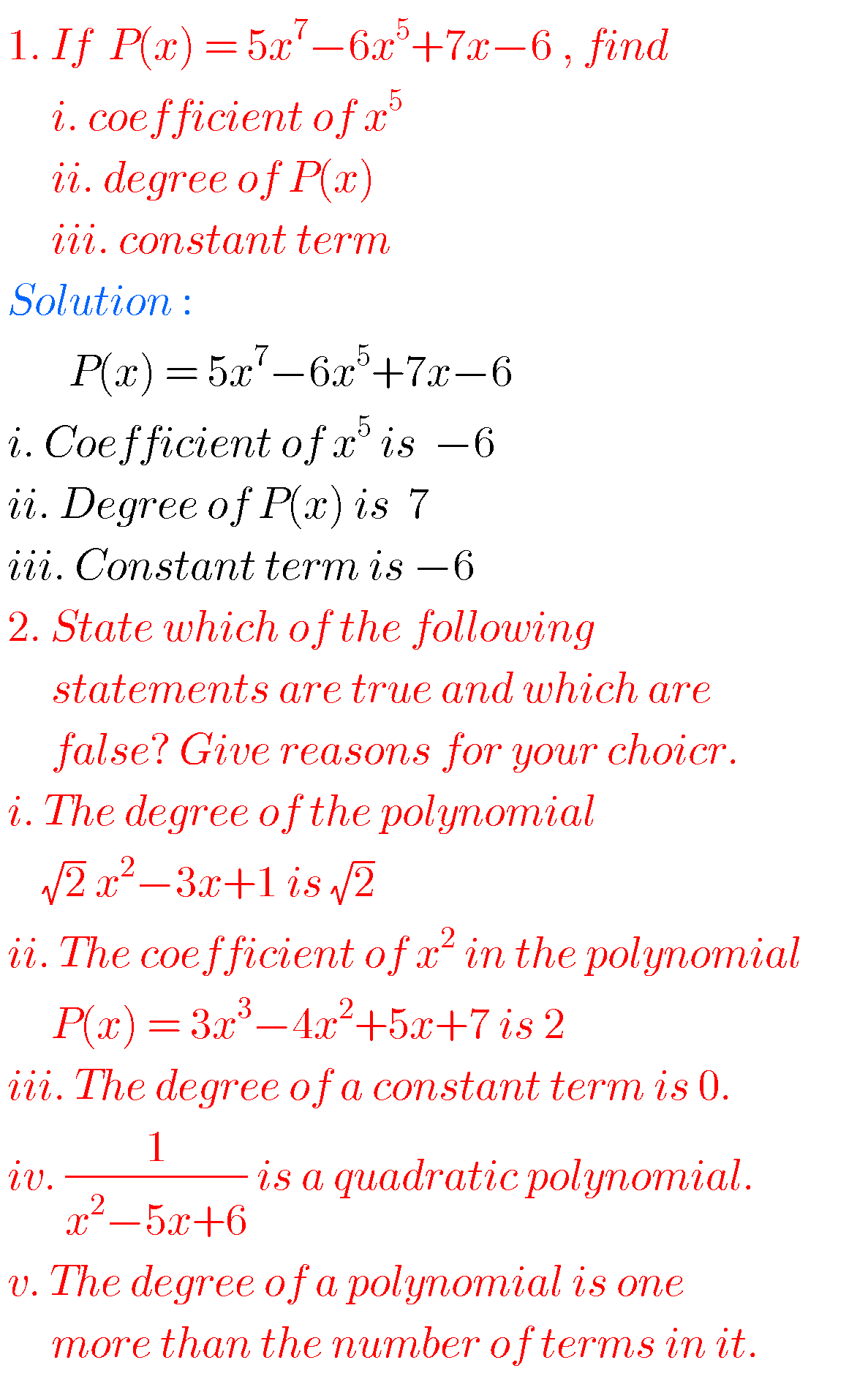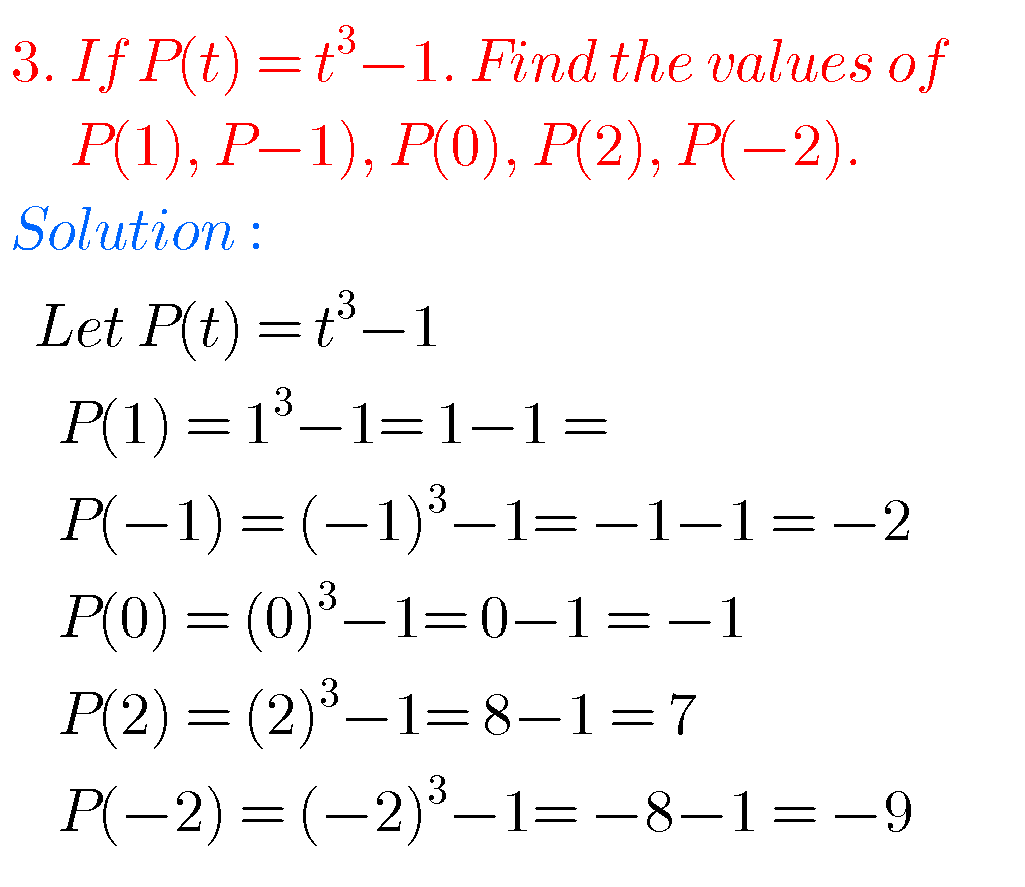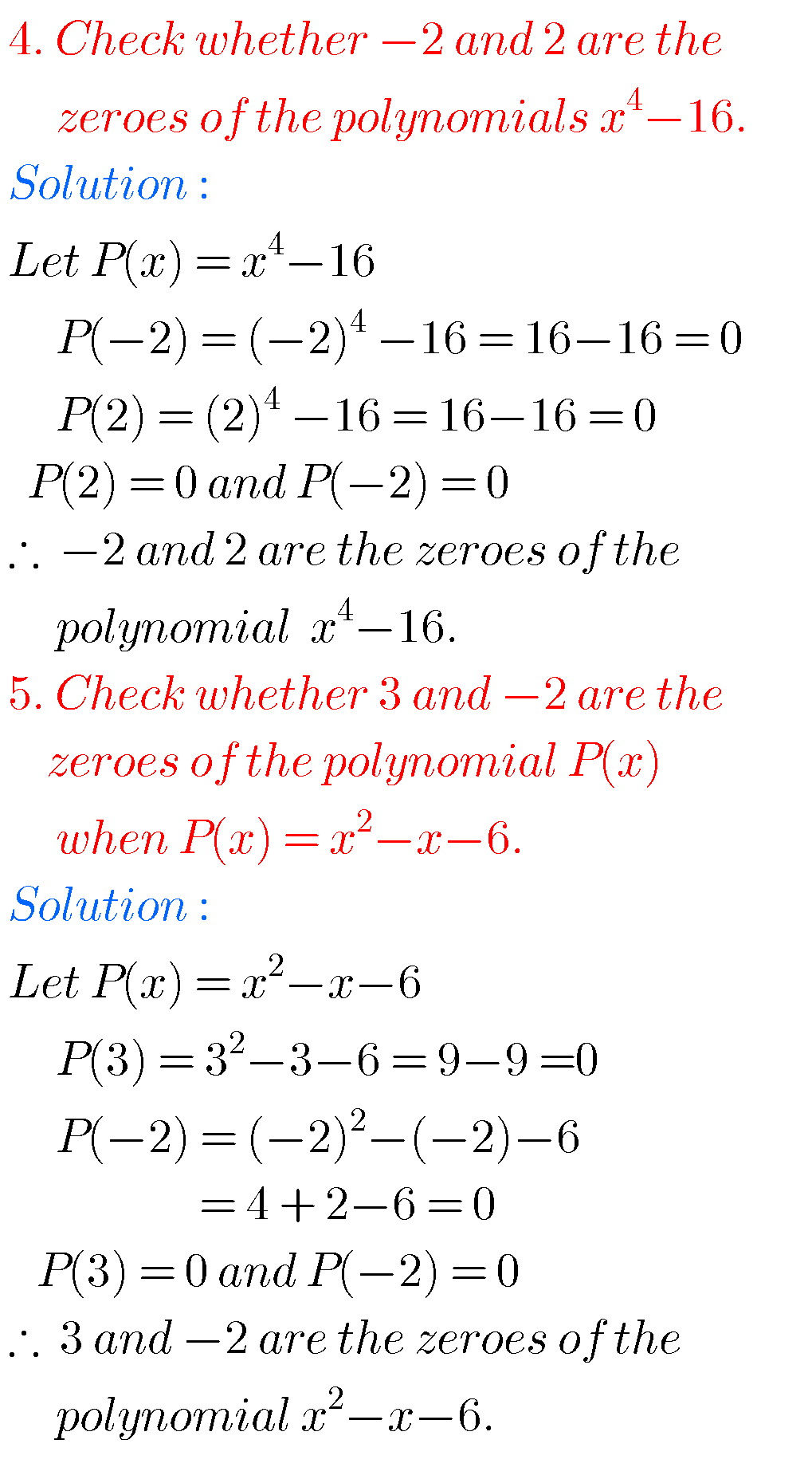## SSC maths polynomials solutions

Exercise 3.2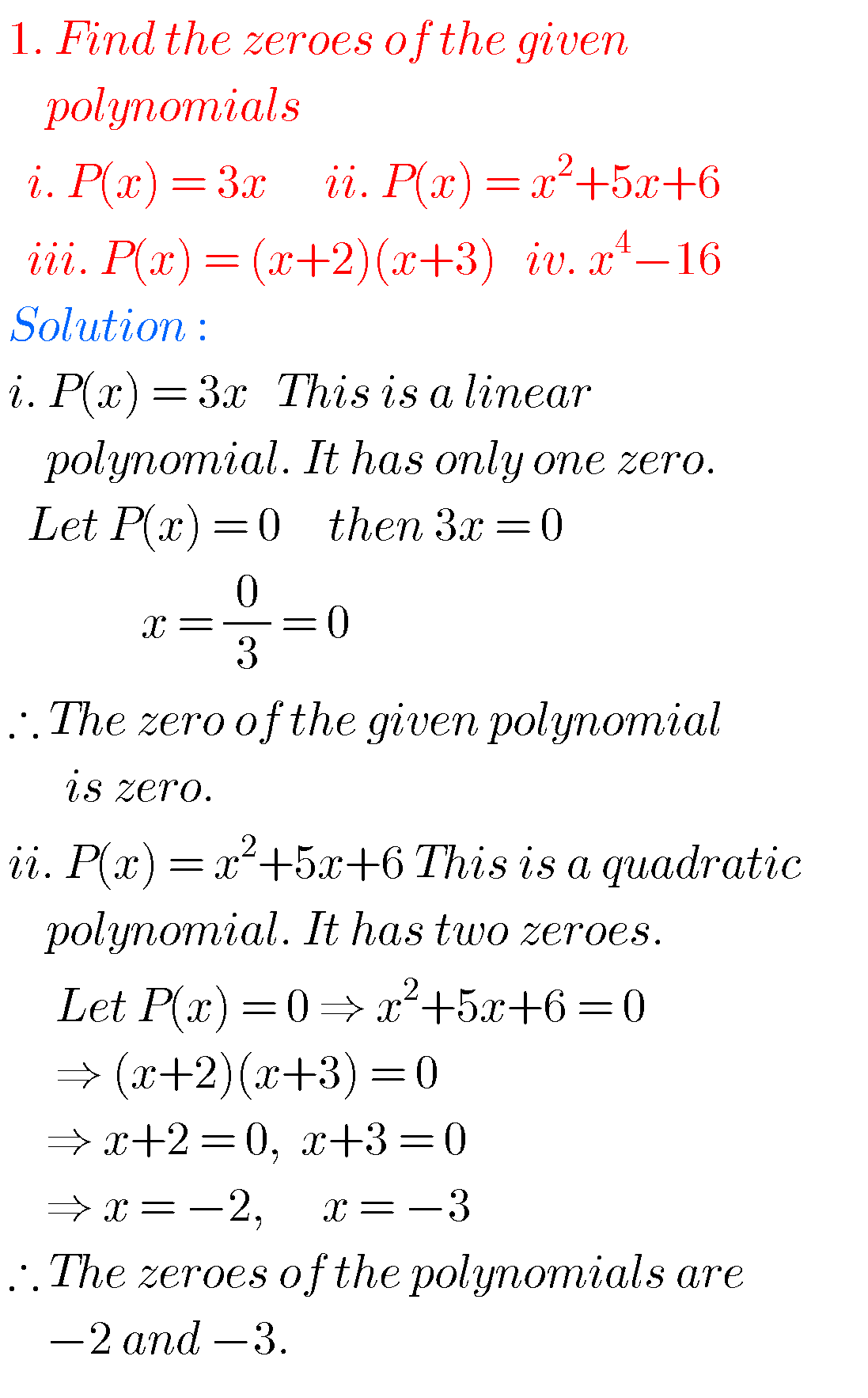##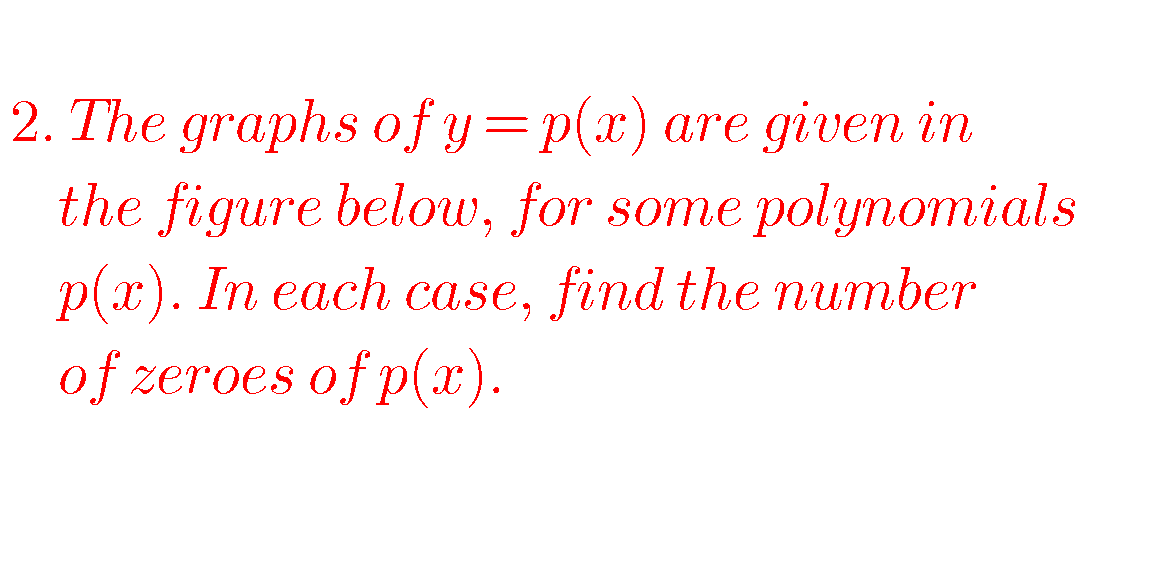iiii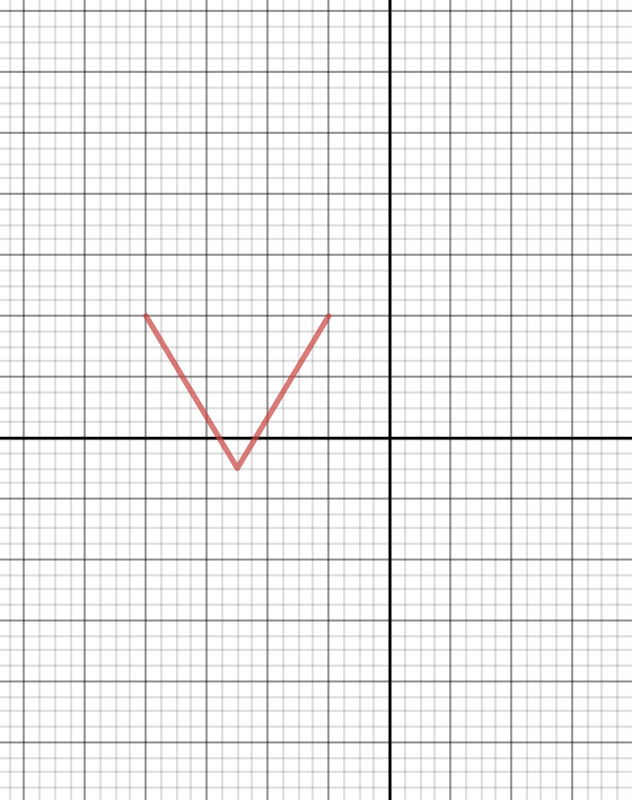iv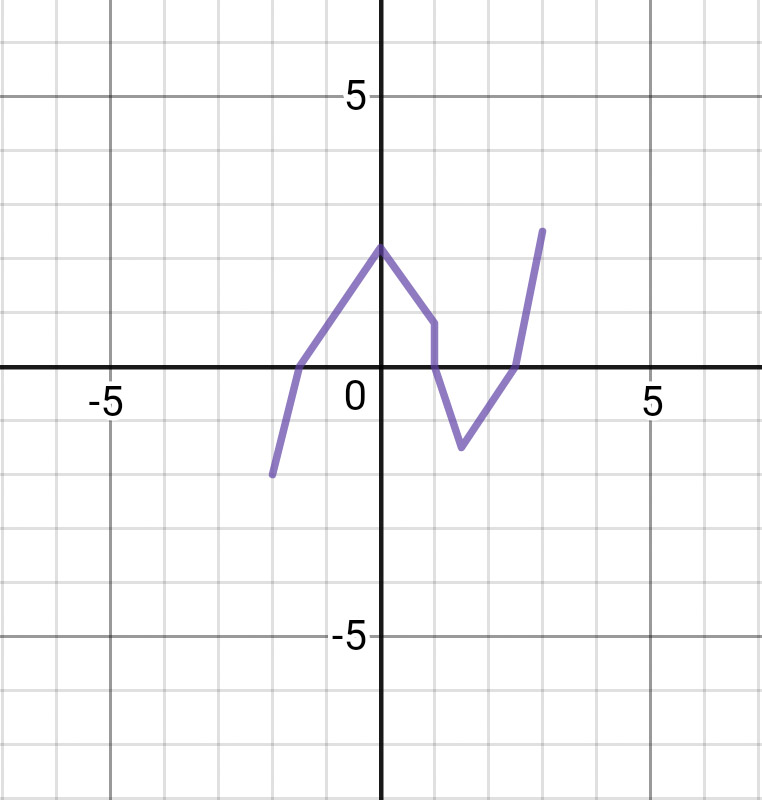Graphs : i, ii,   , iv, v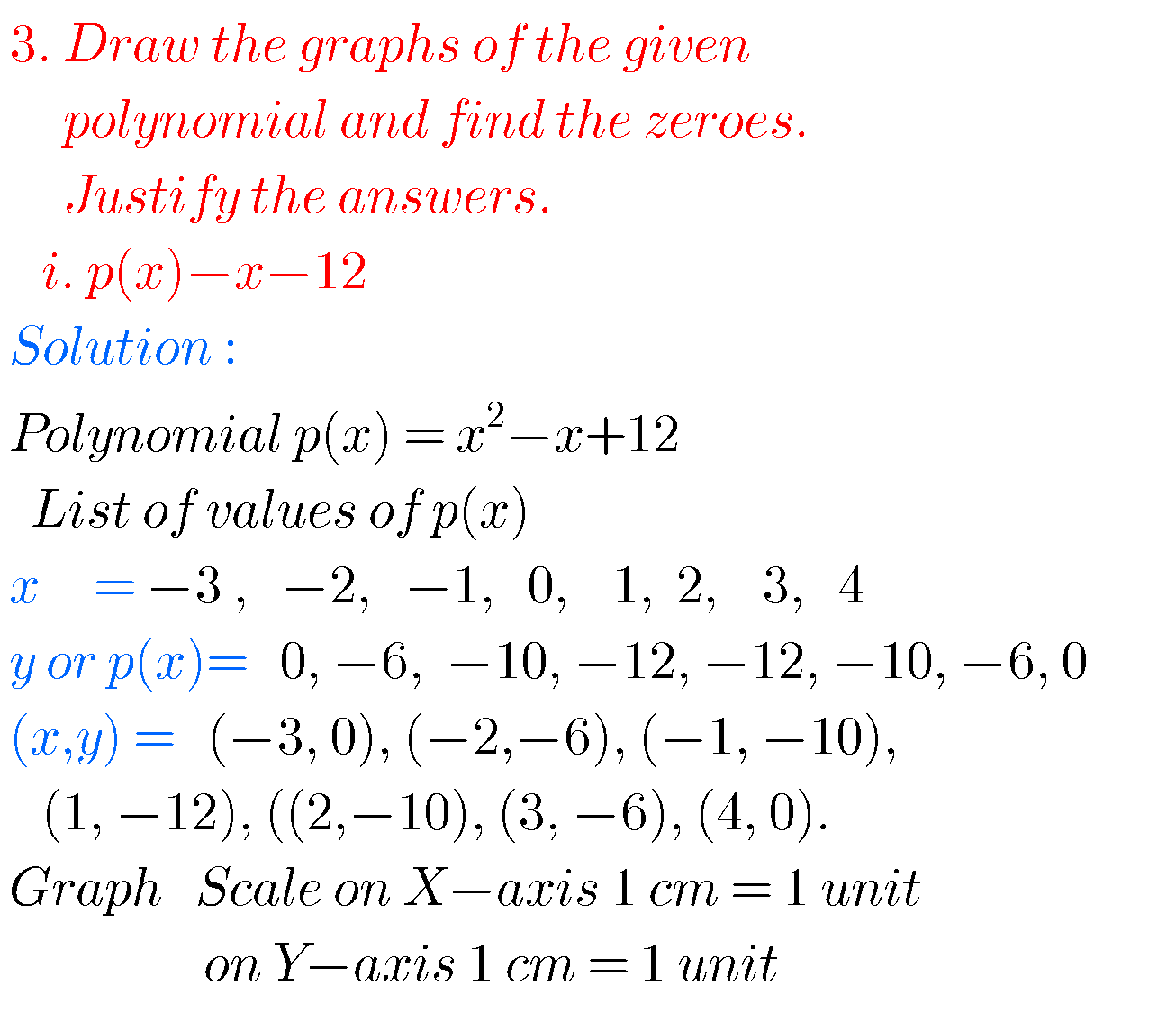Graph i##Graph ii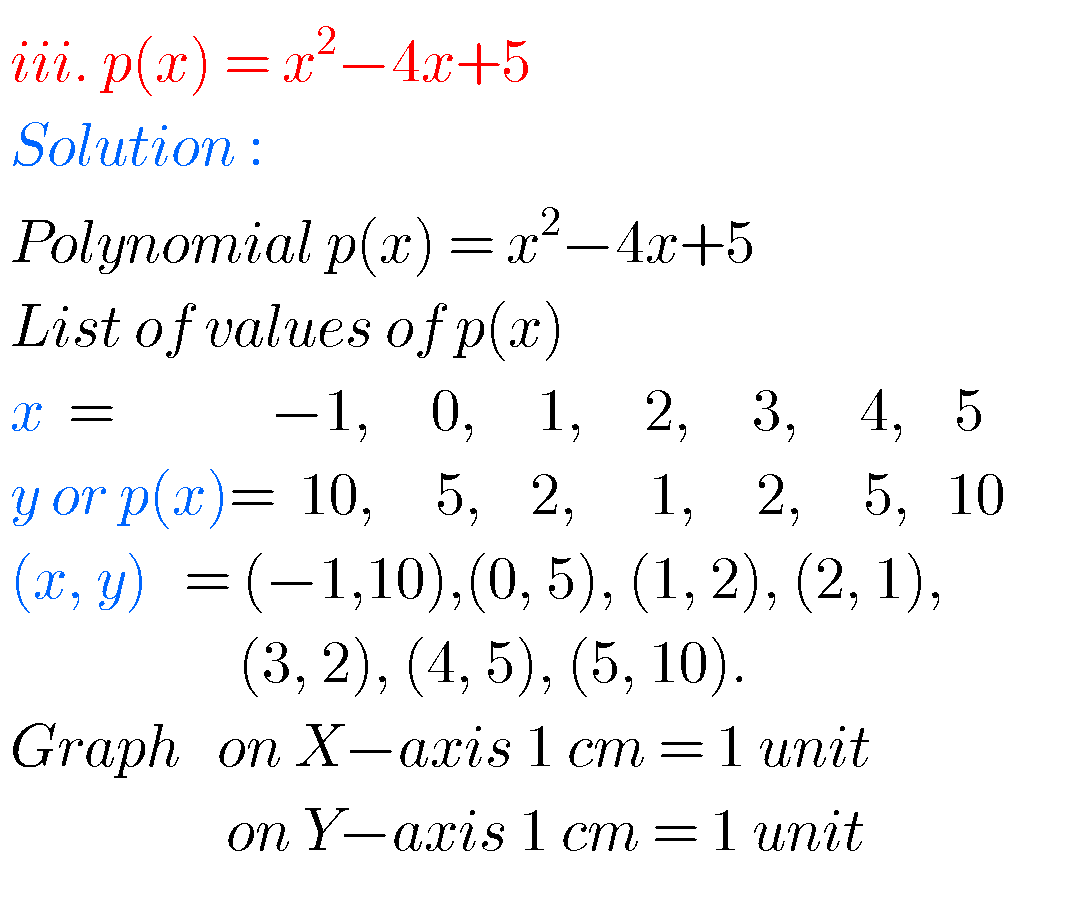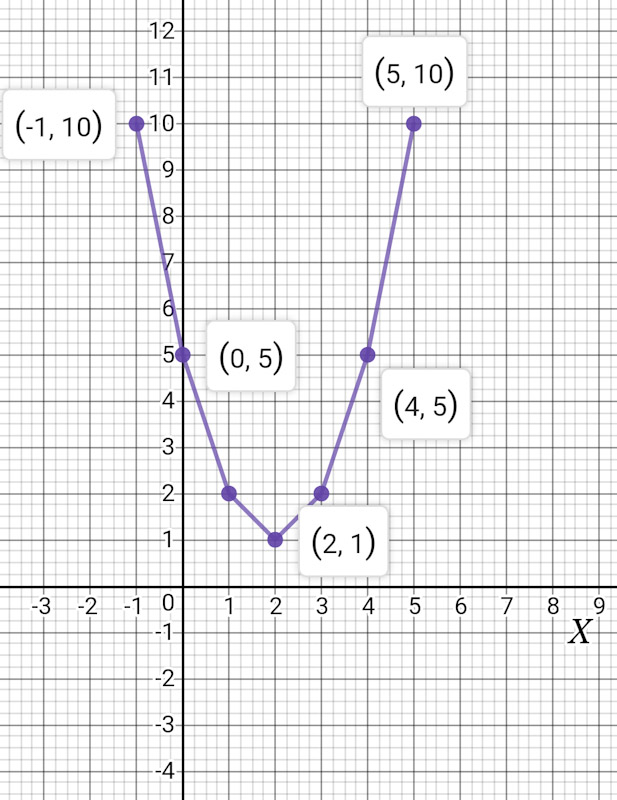Graph iii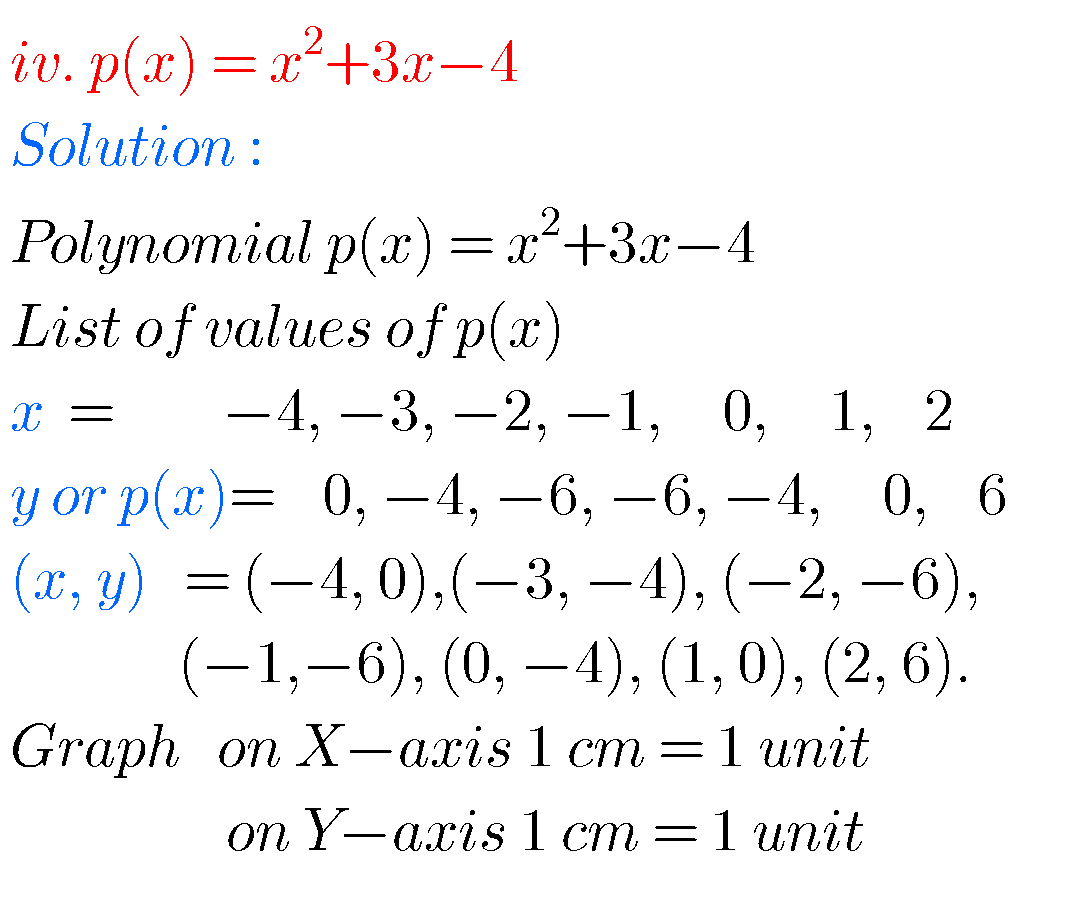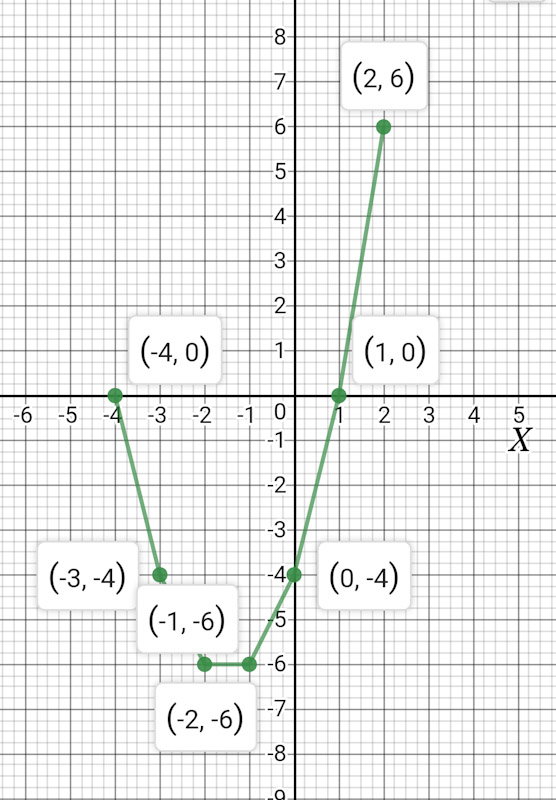Graph iv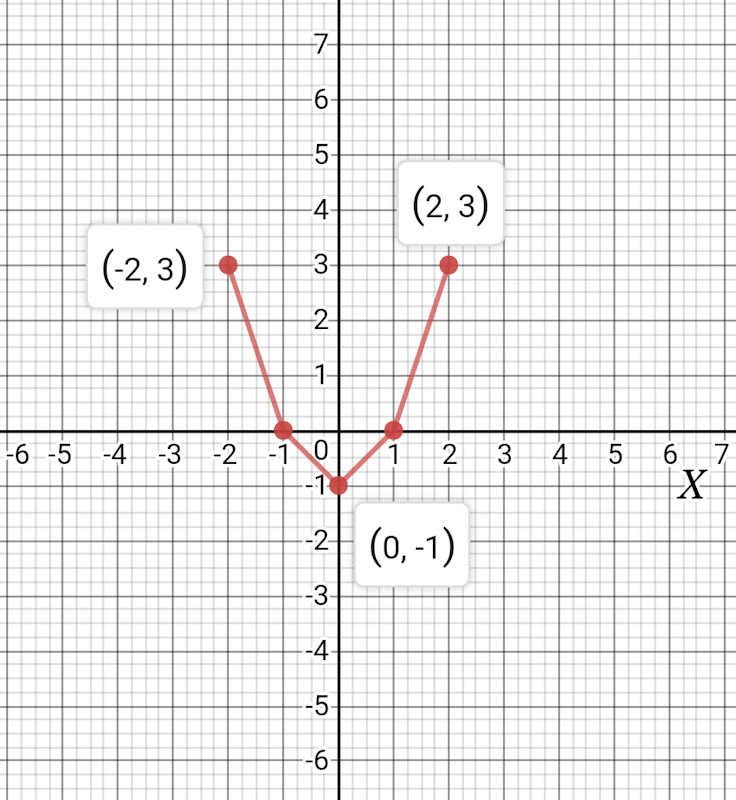Graph v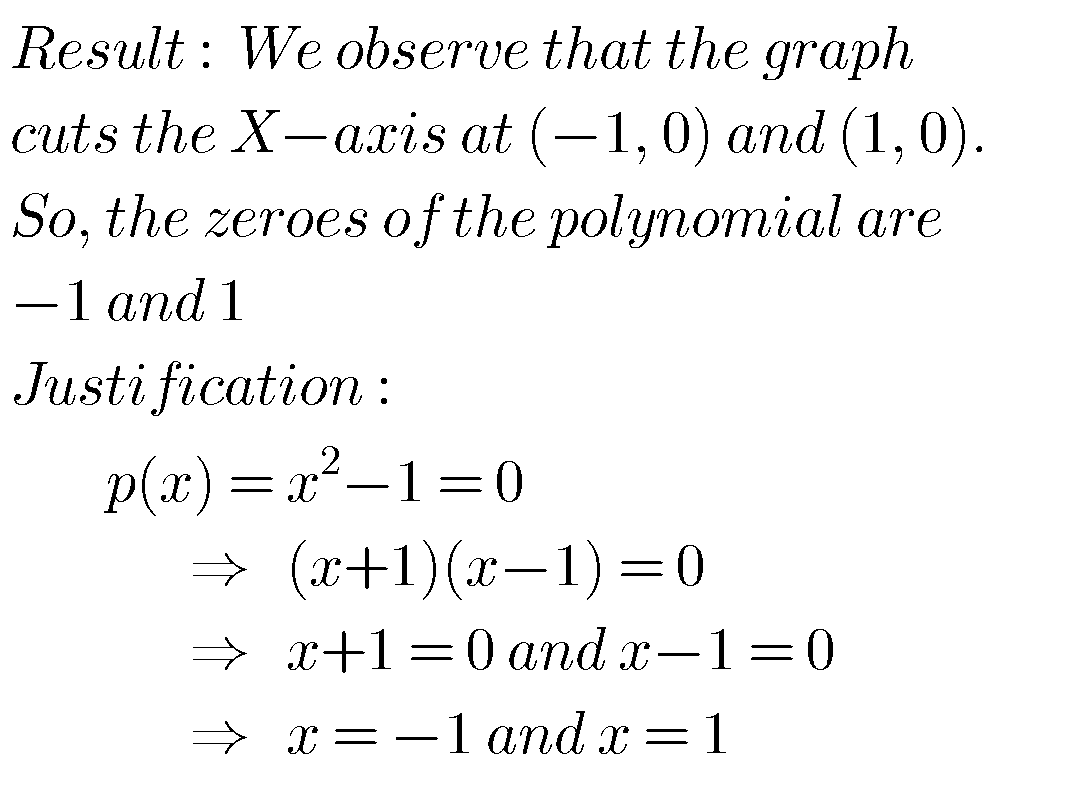## Maths class X polynomials solutions

Exercise 3.3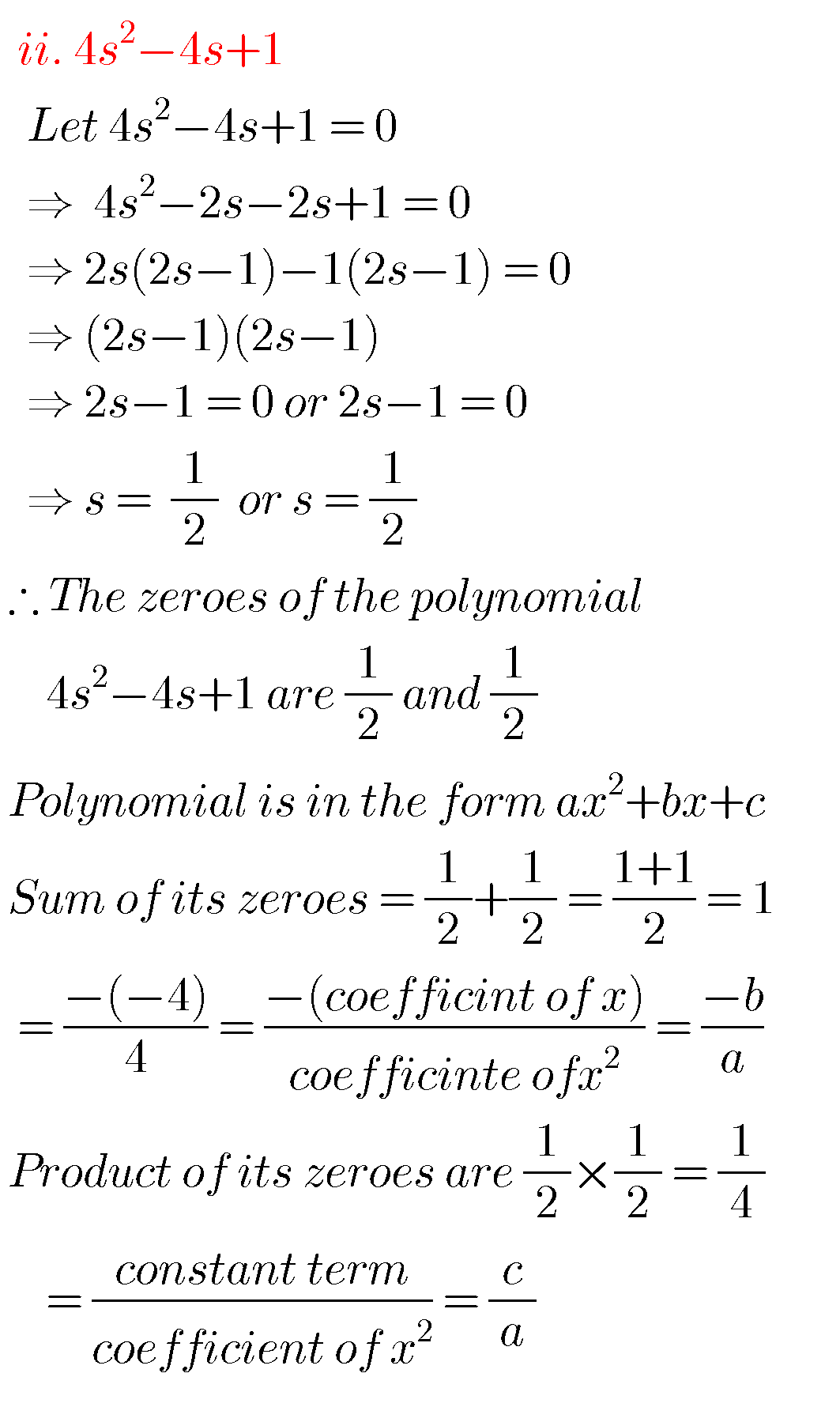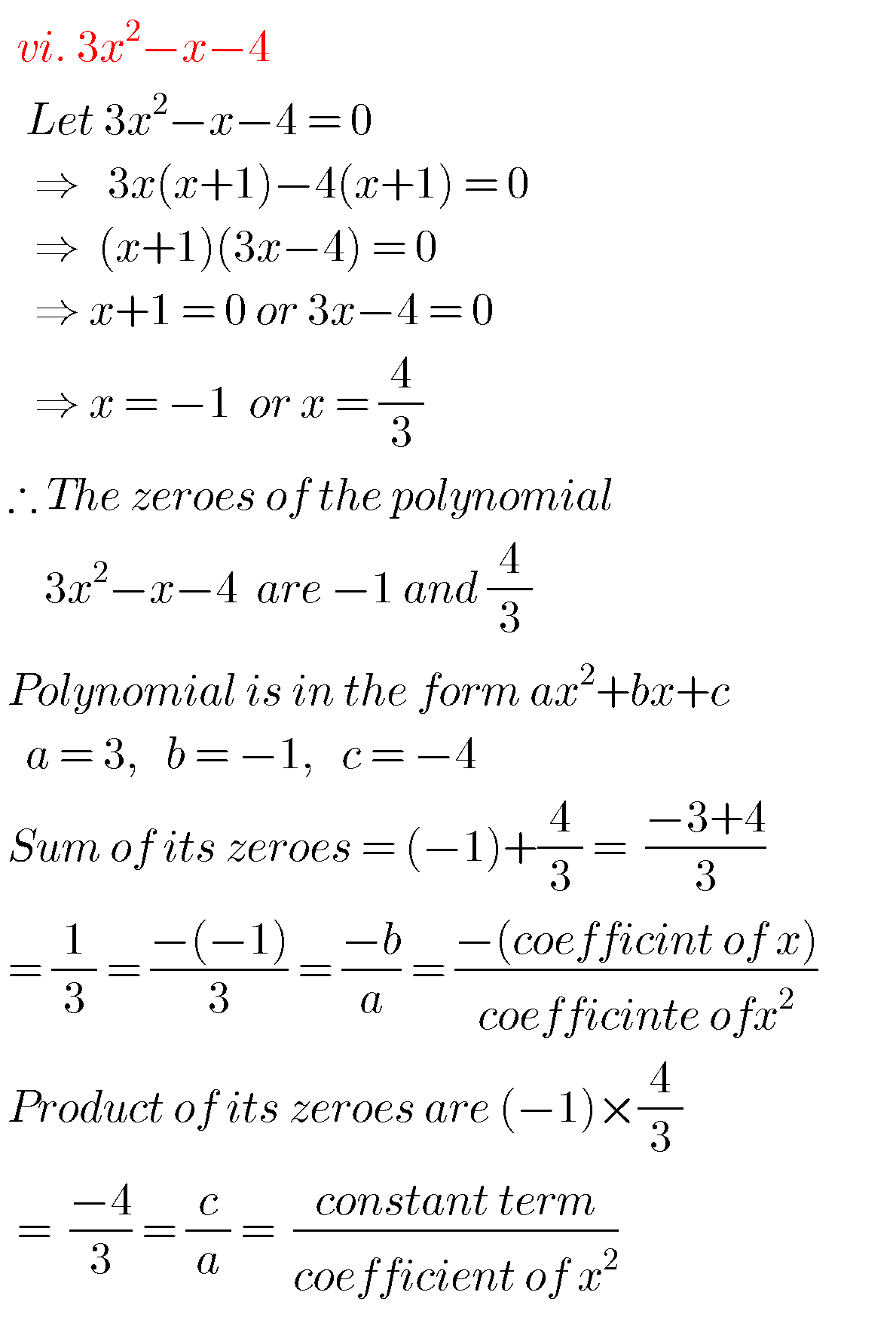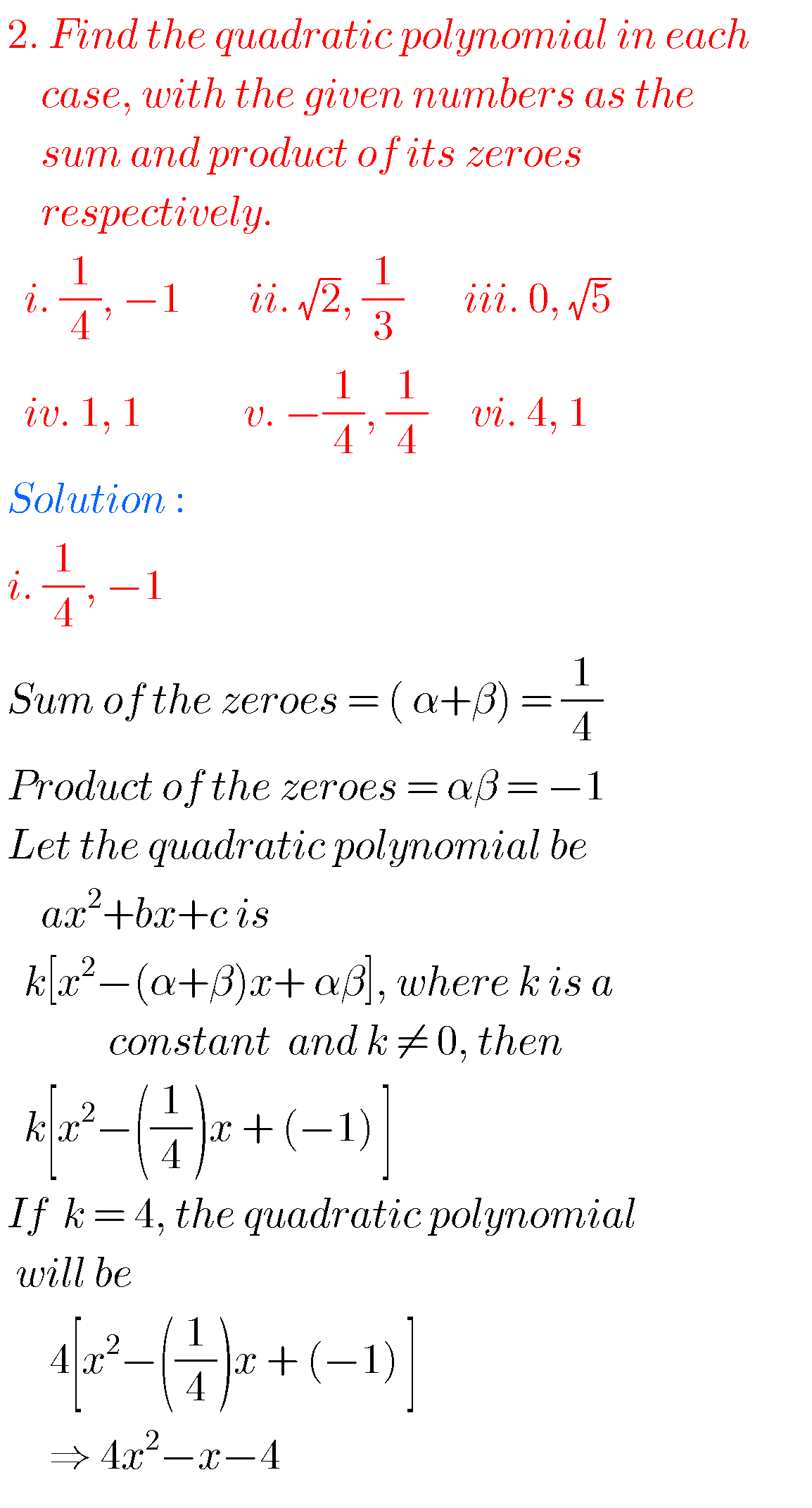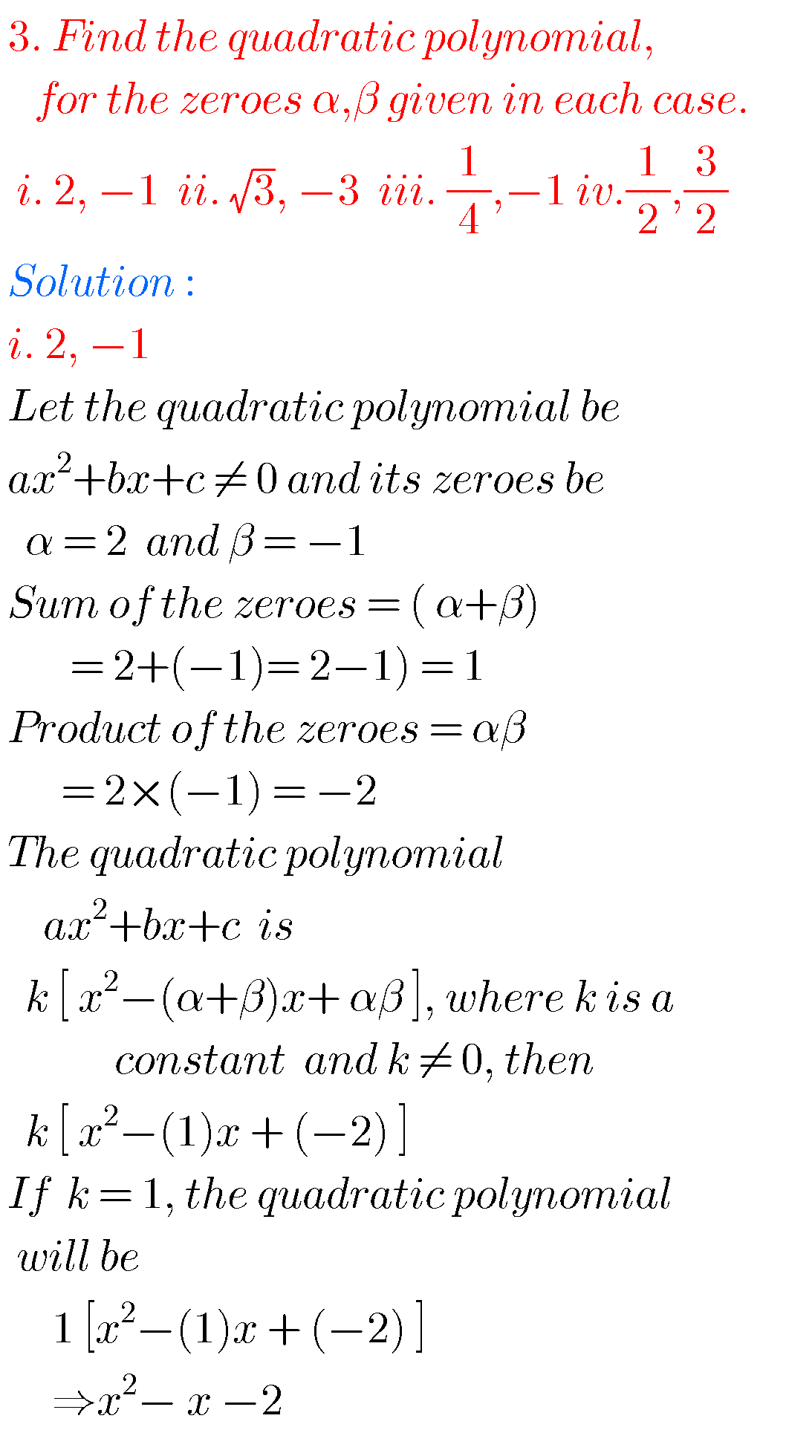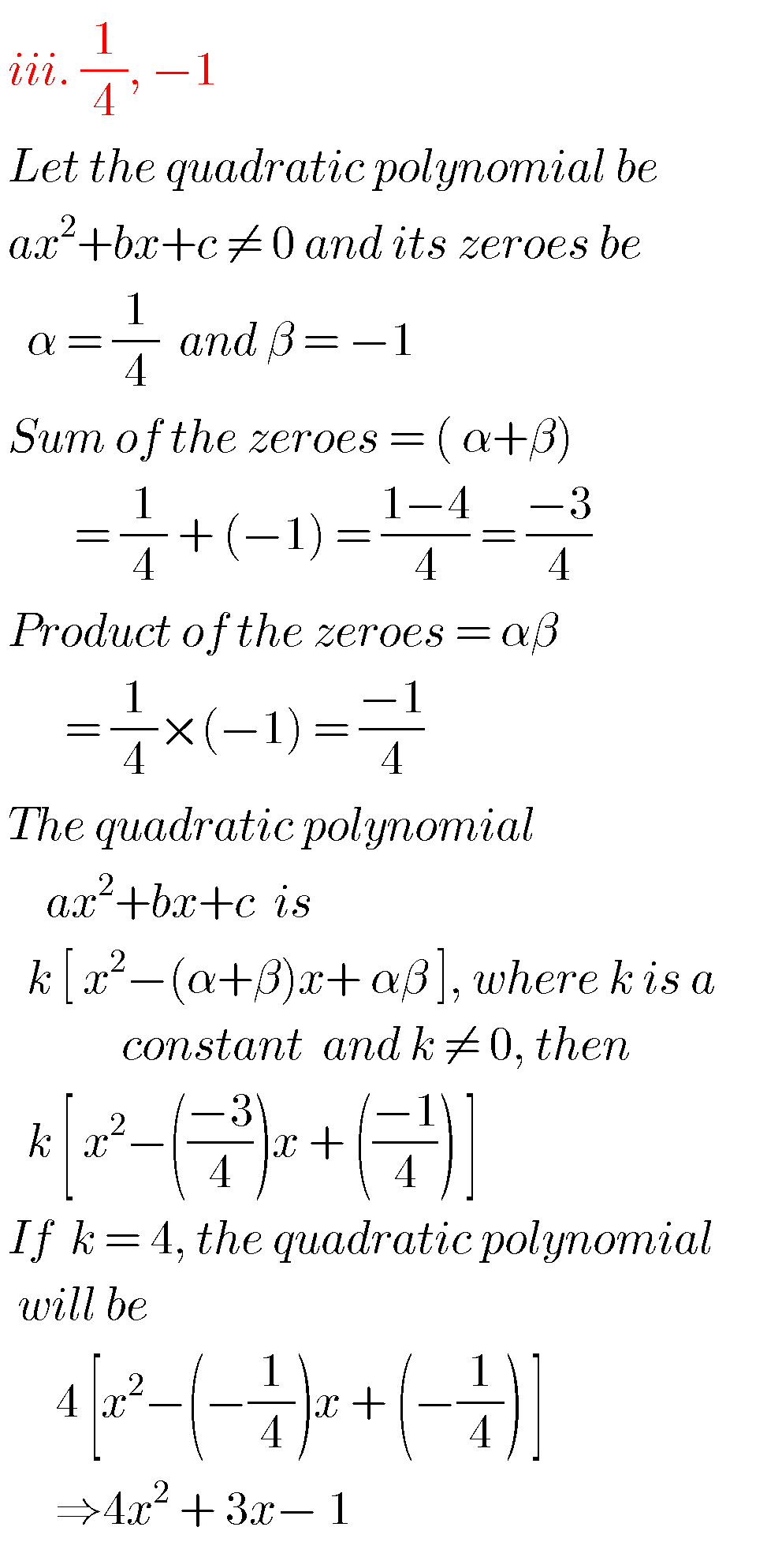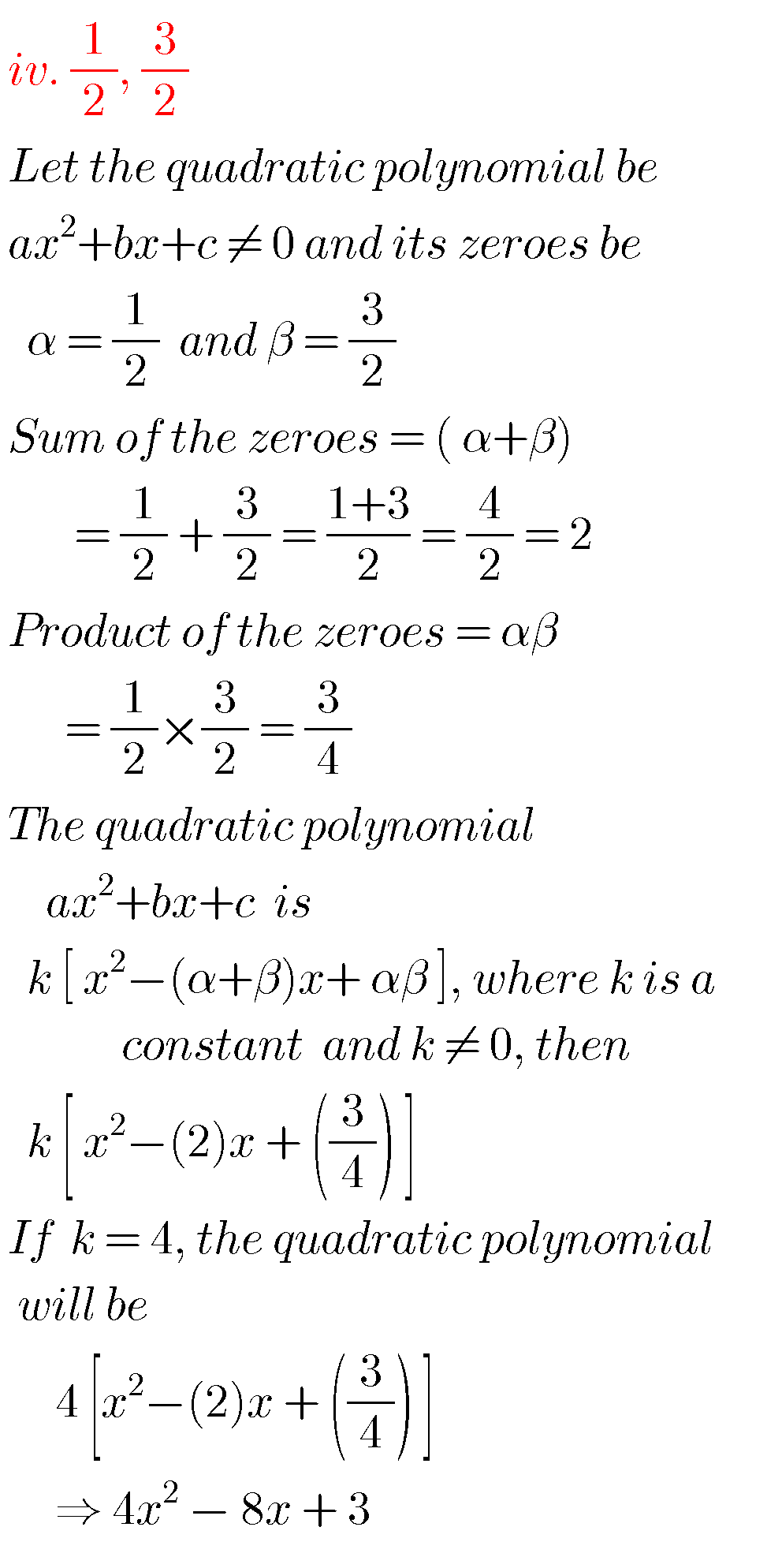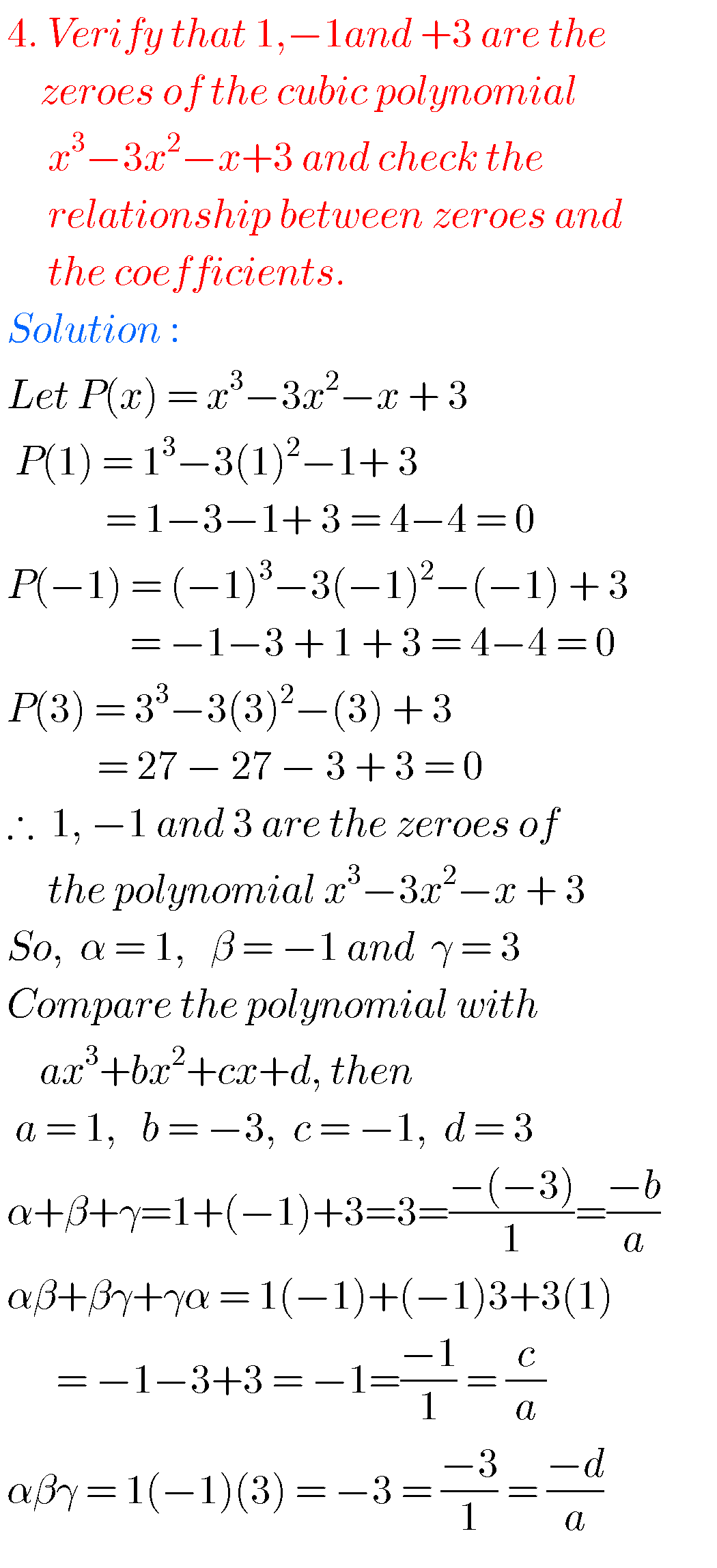### 10th class maths polynomials solutions

Exercise 3.4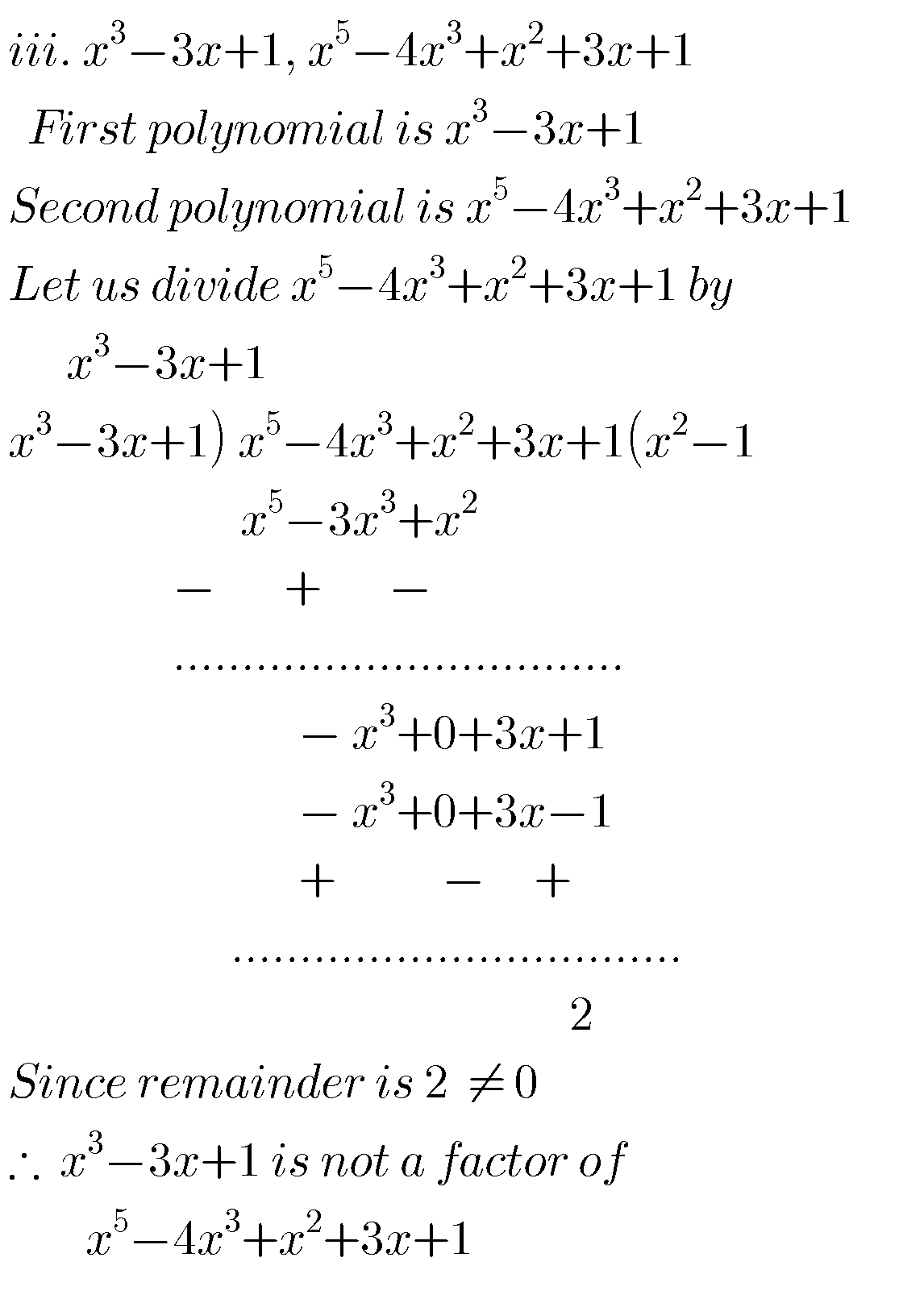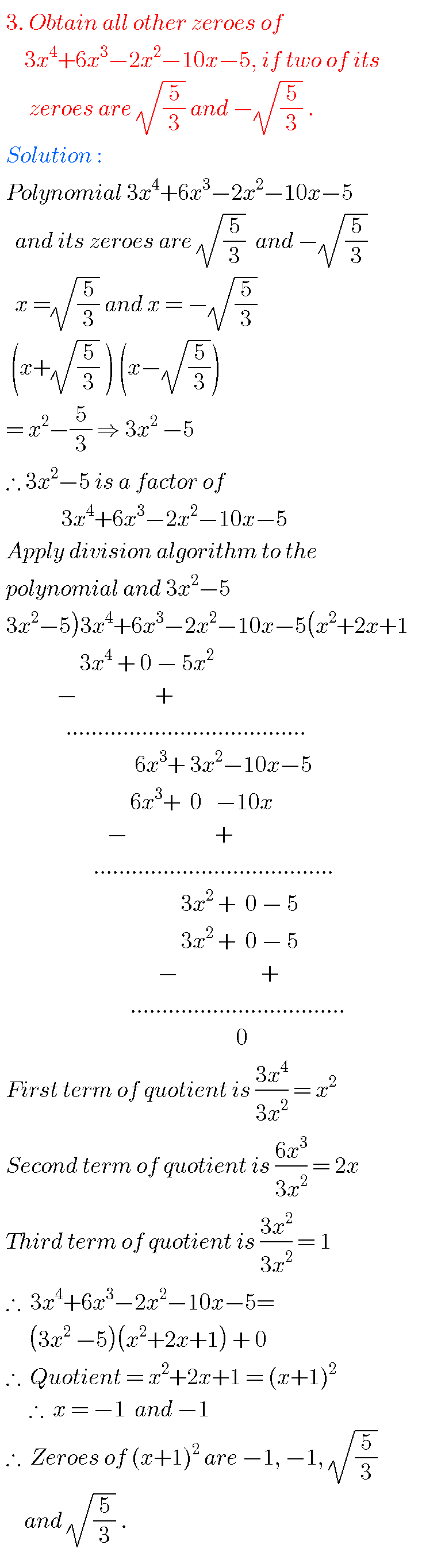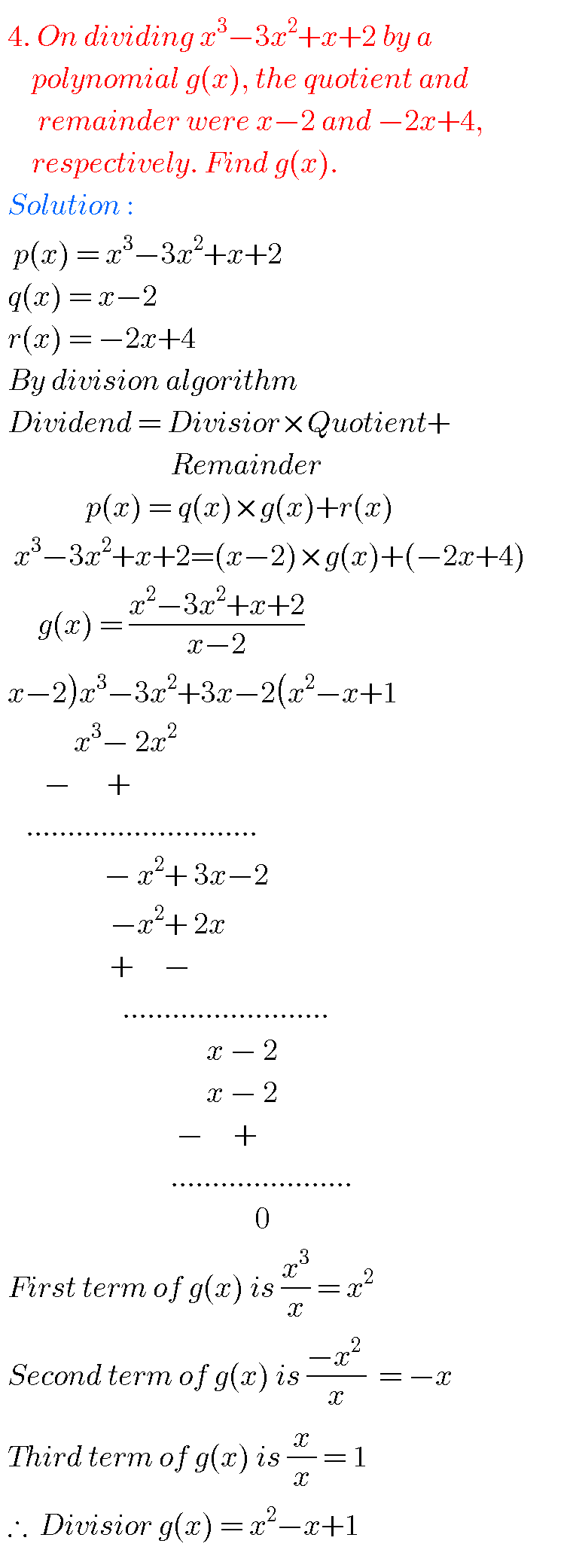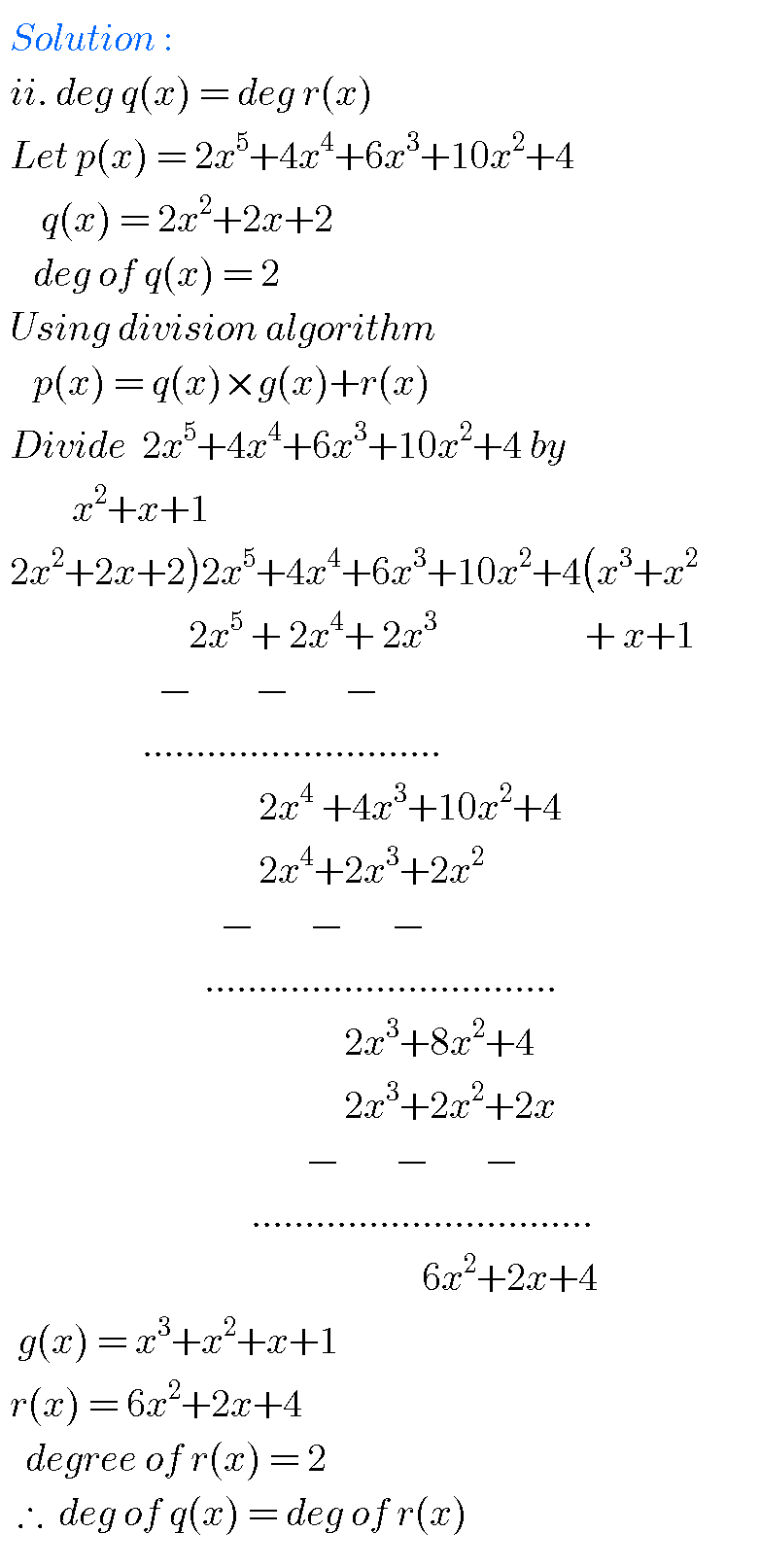### Polynomials class 10 maths

Optinal exercise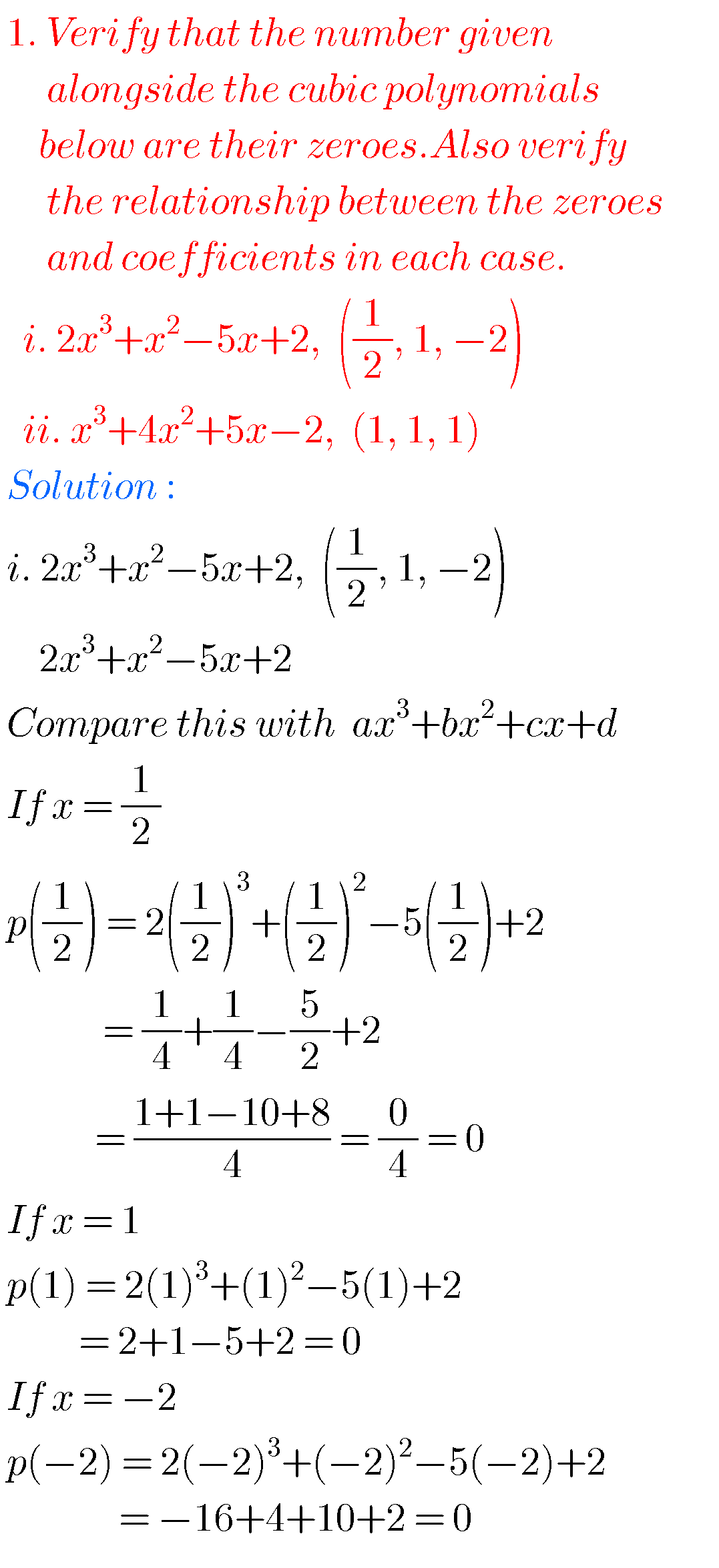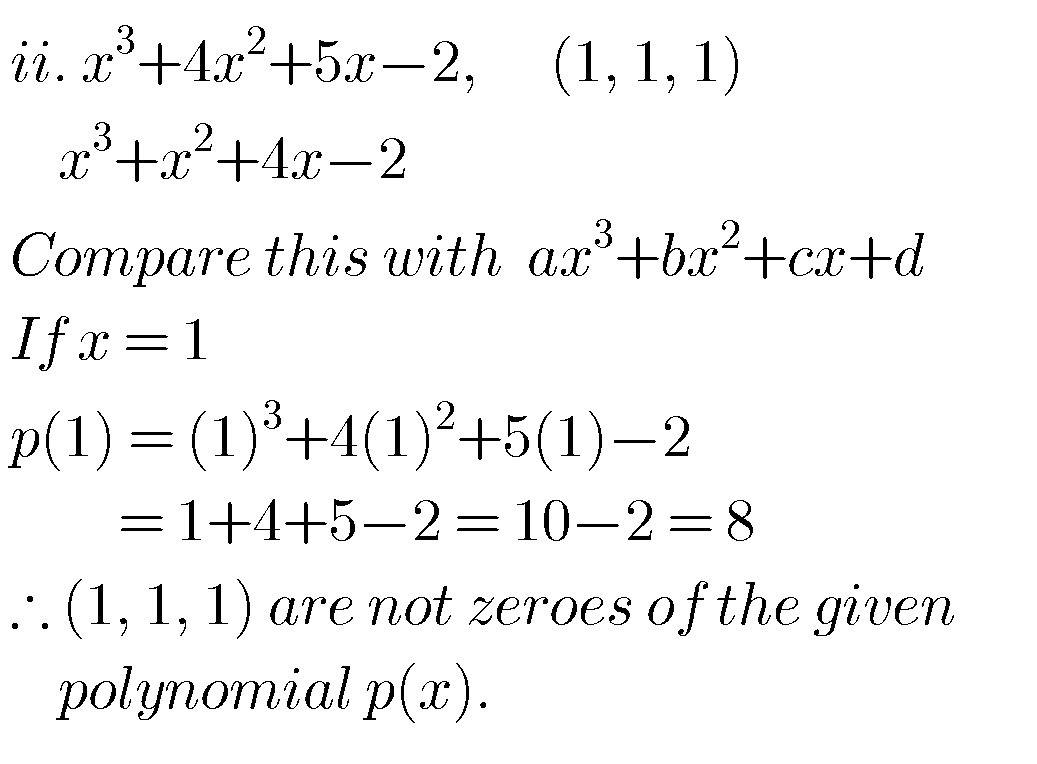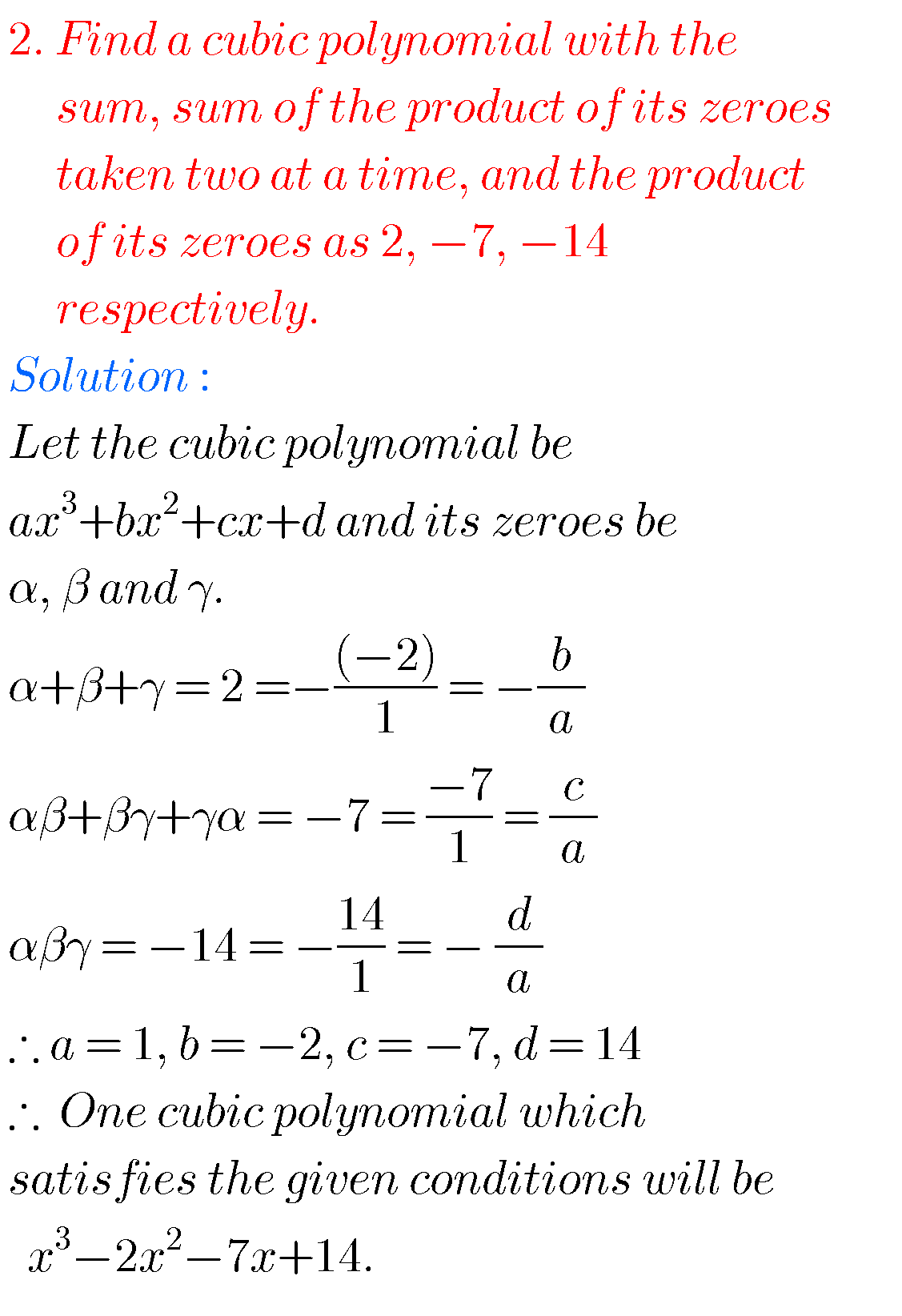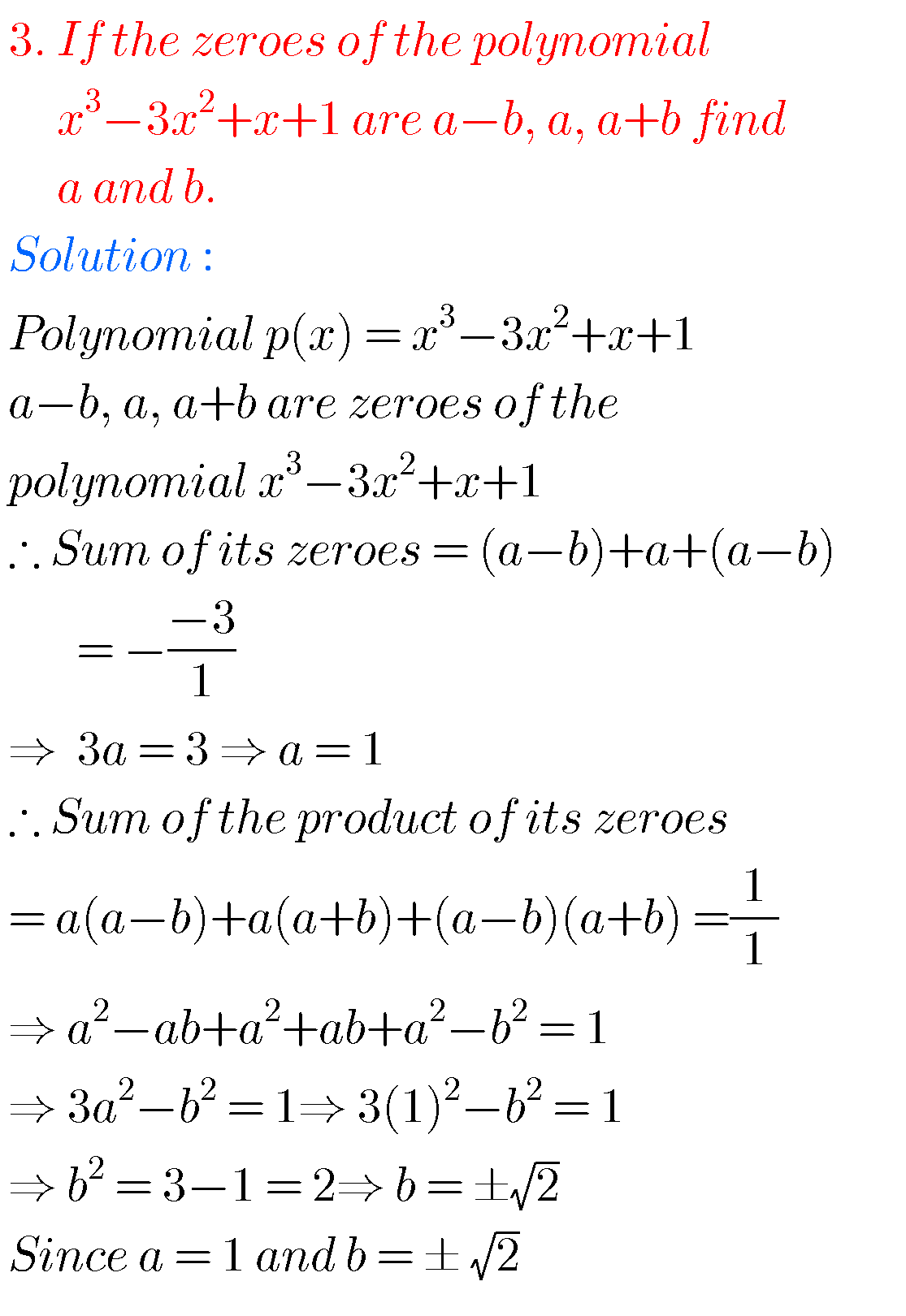Some more solutions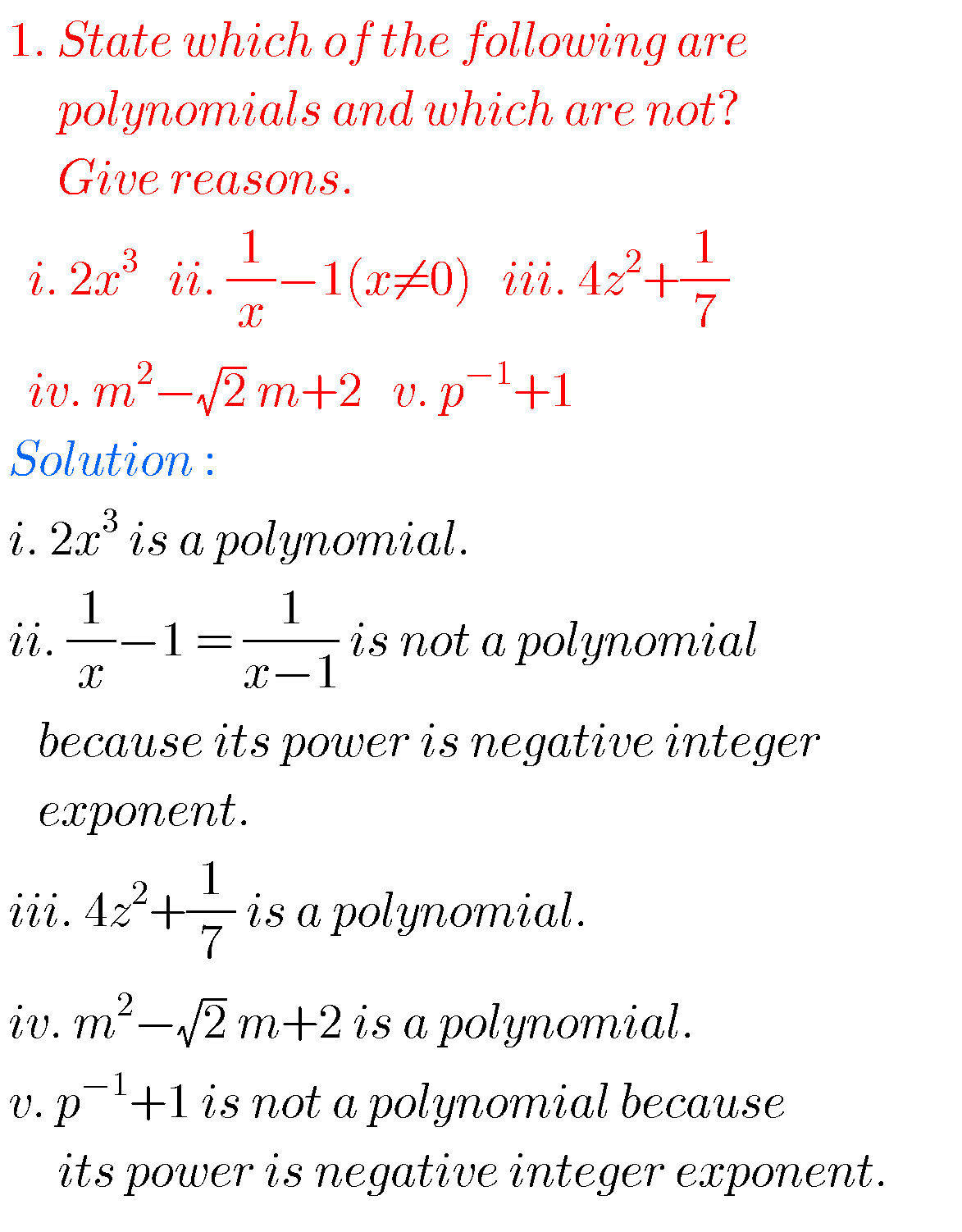4.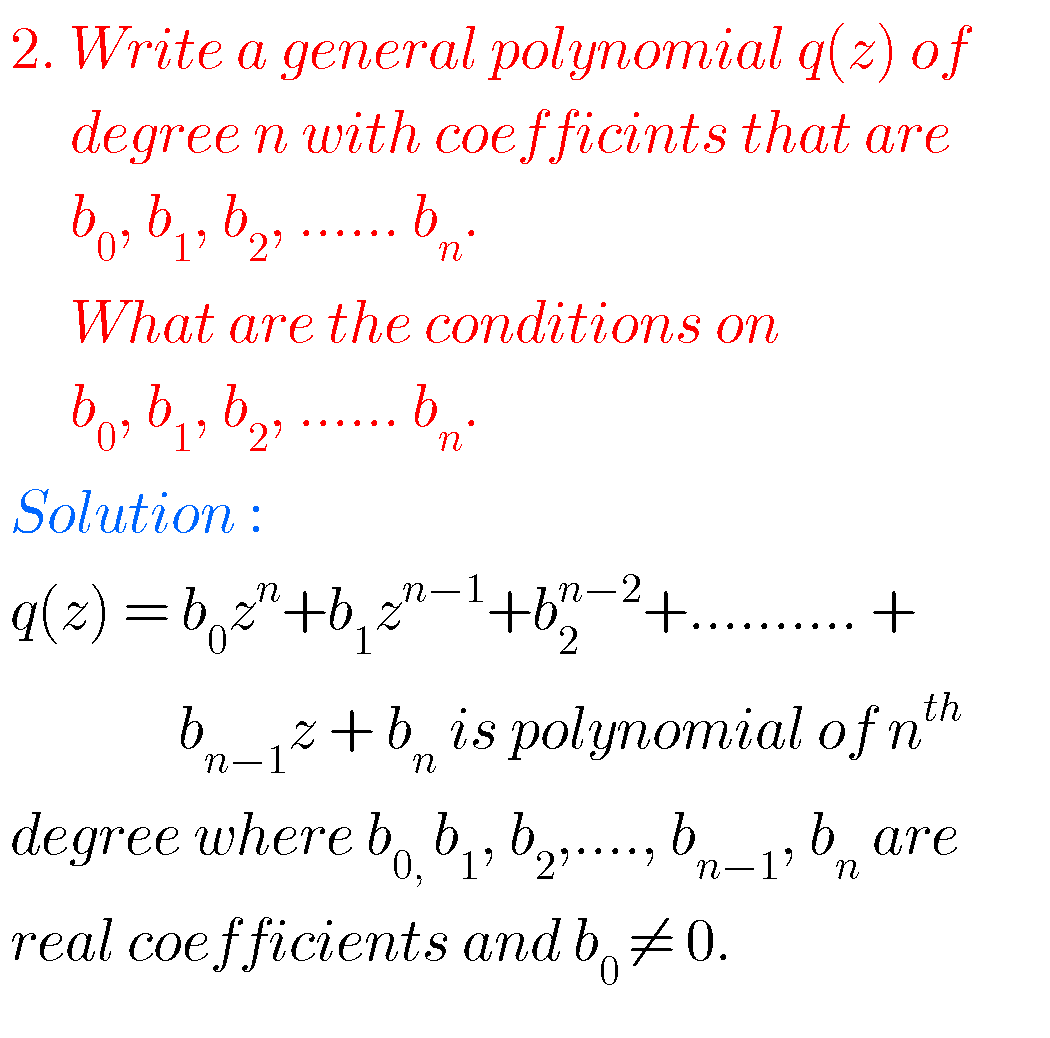GraphGraphGraph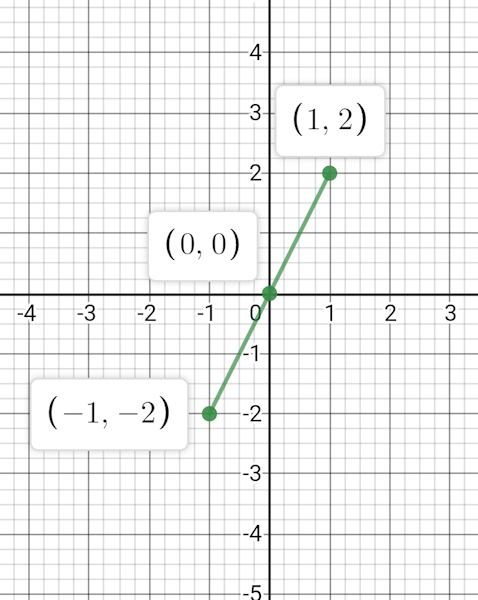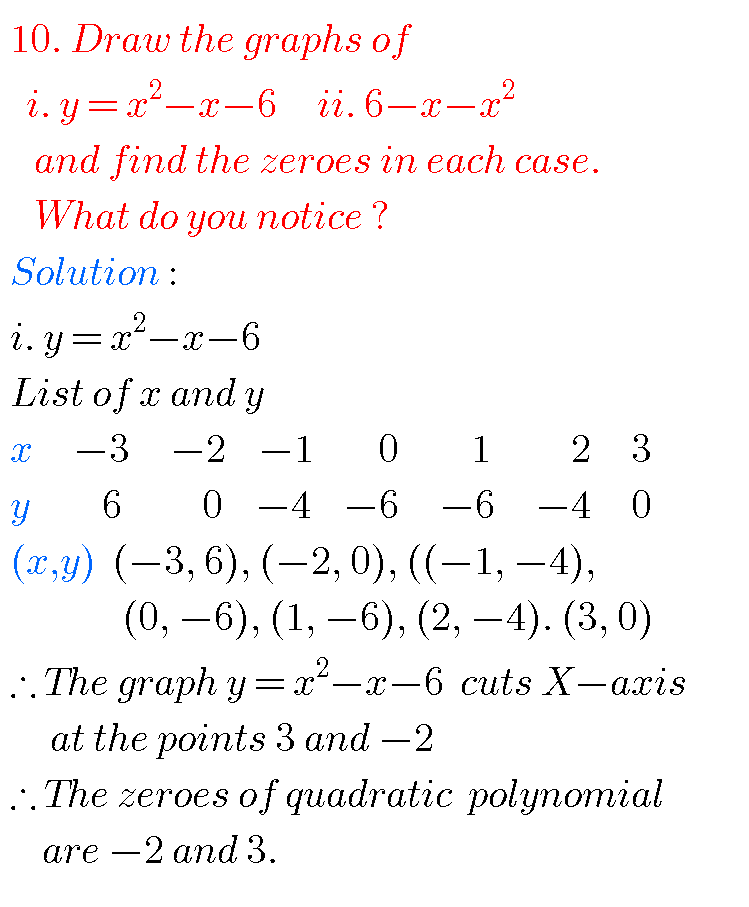GraphGraph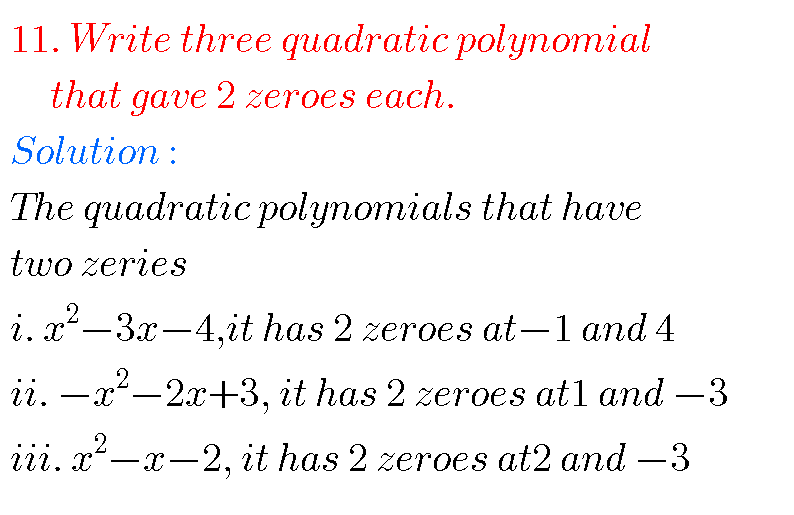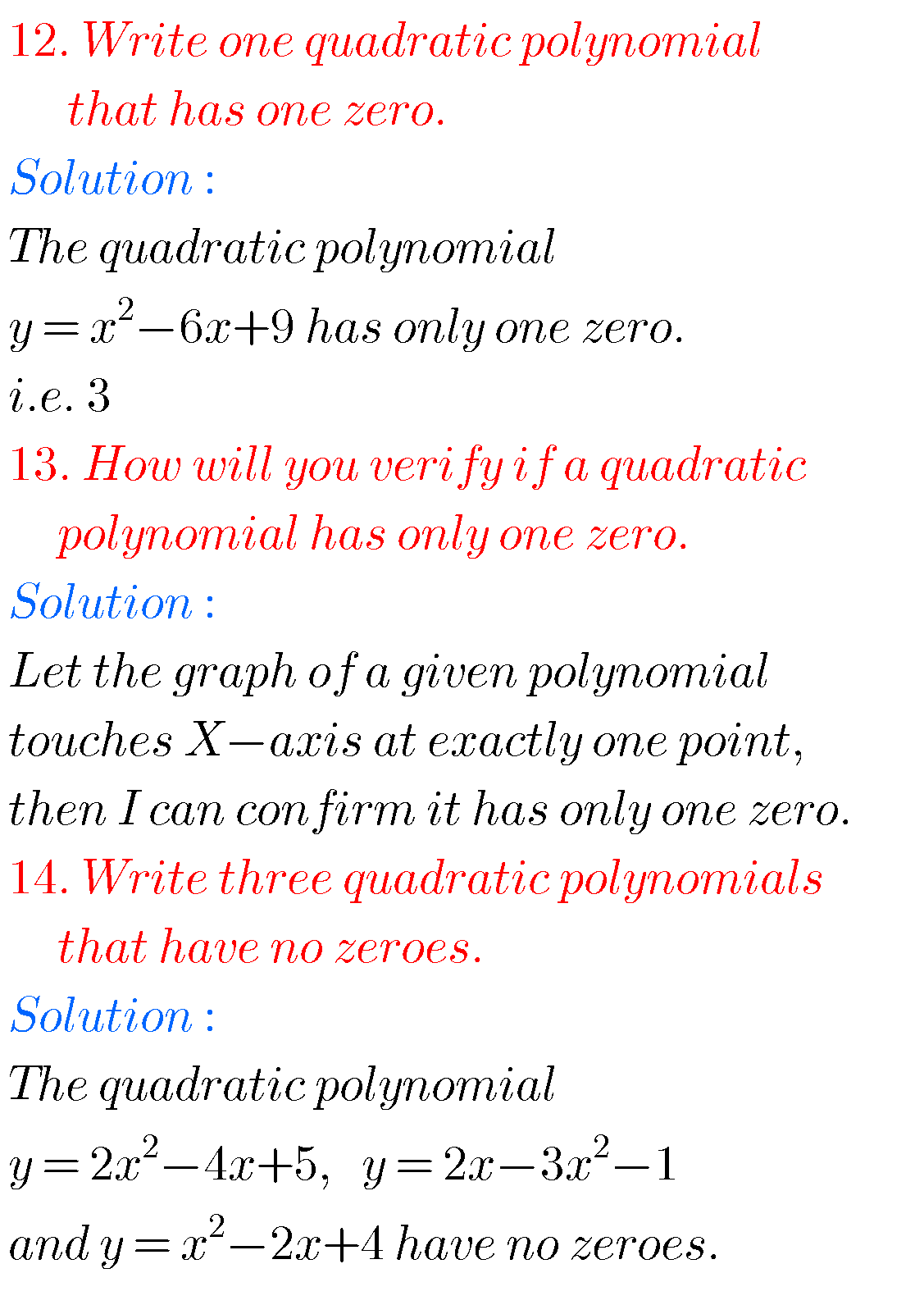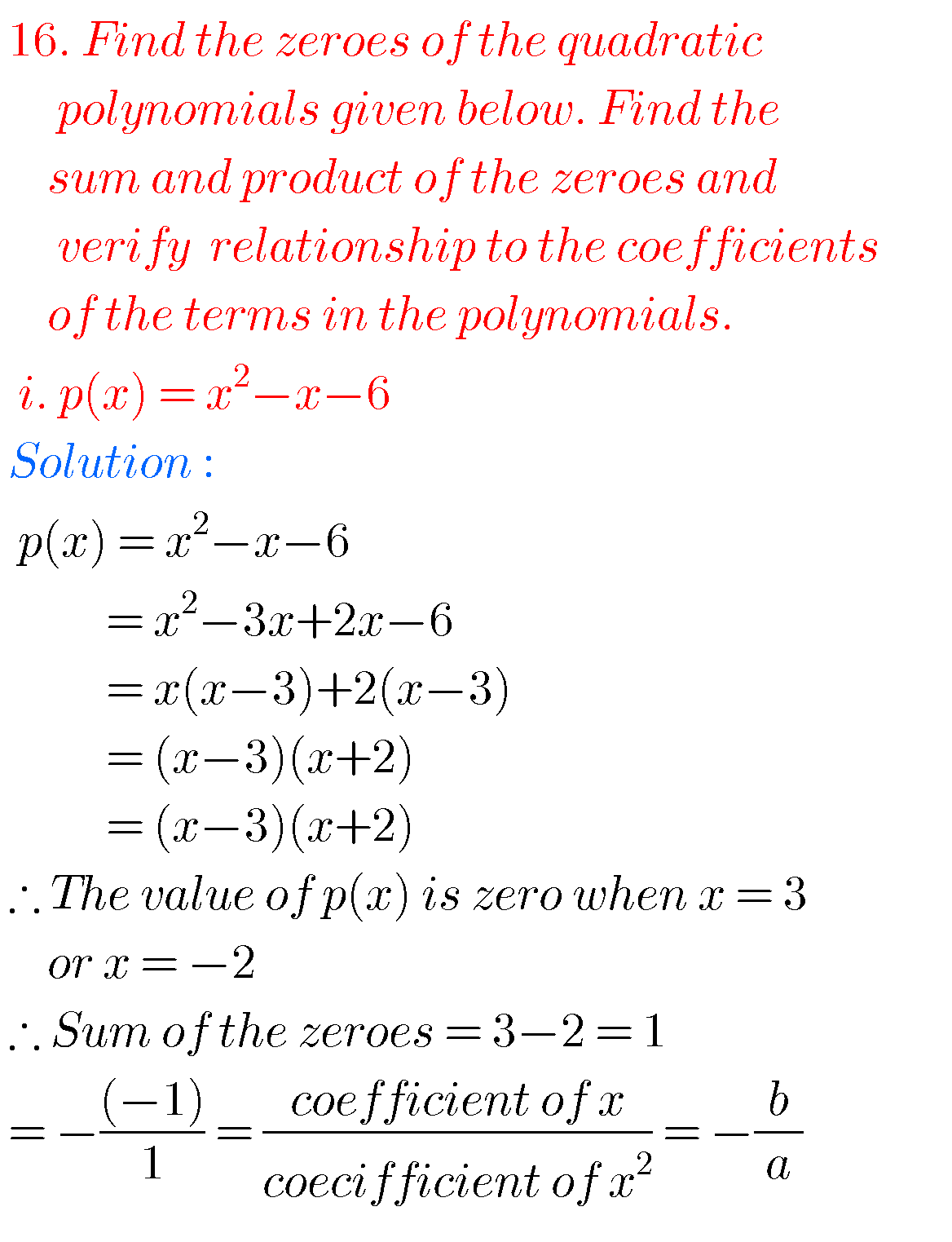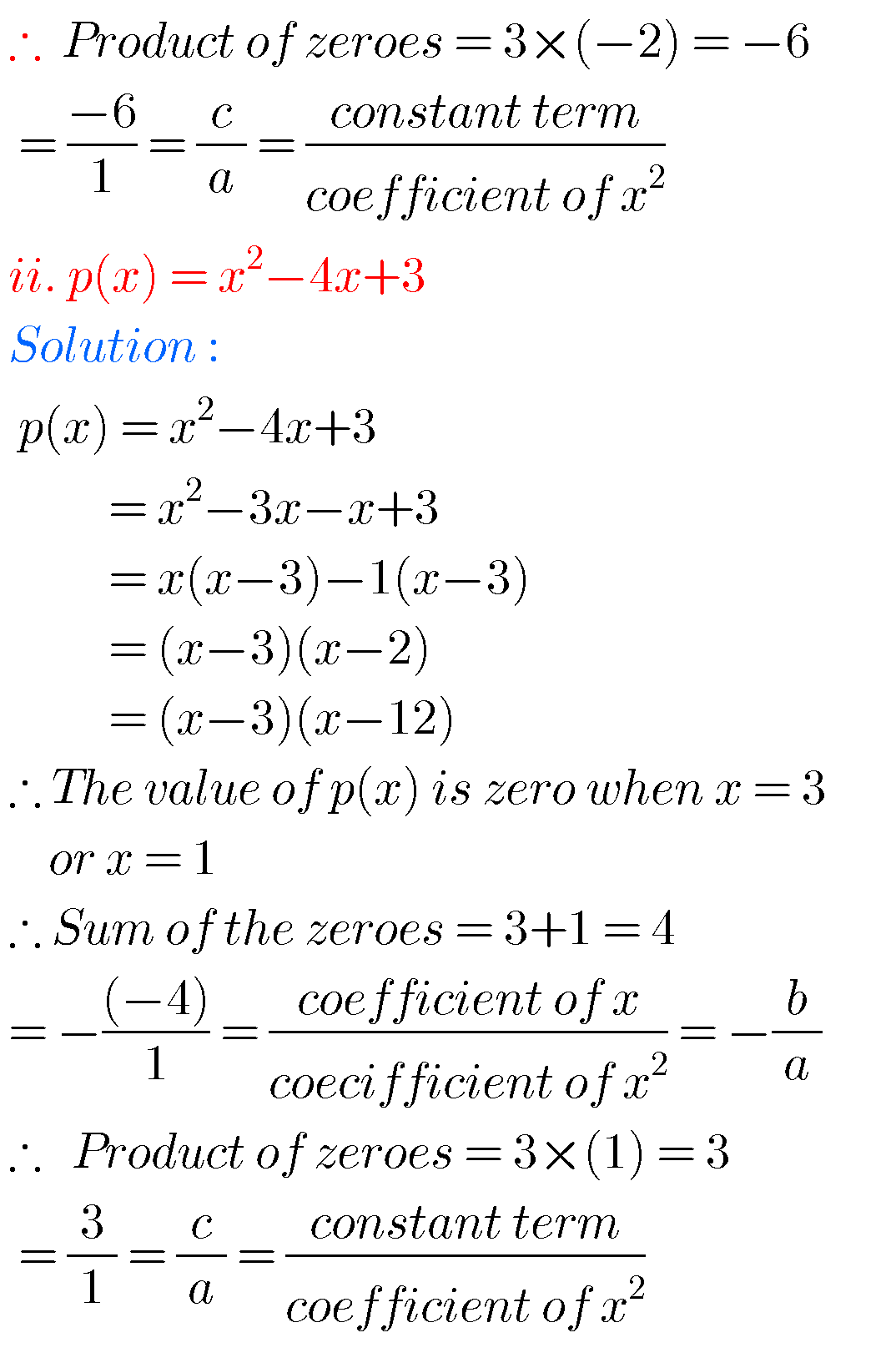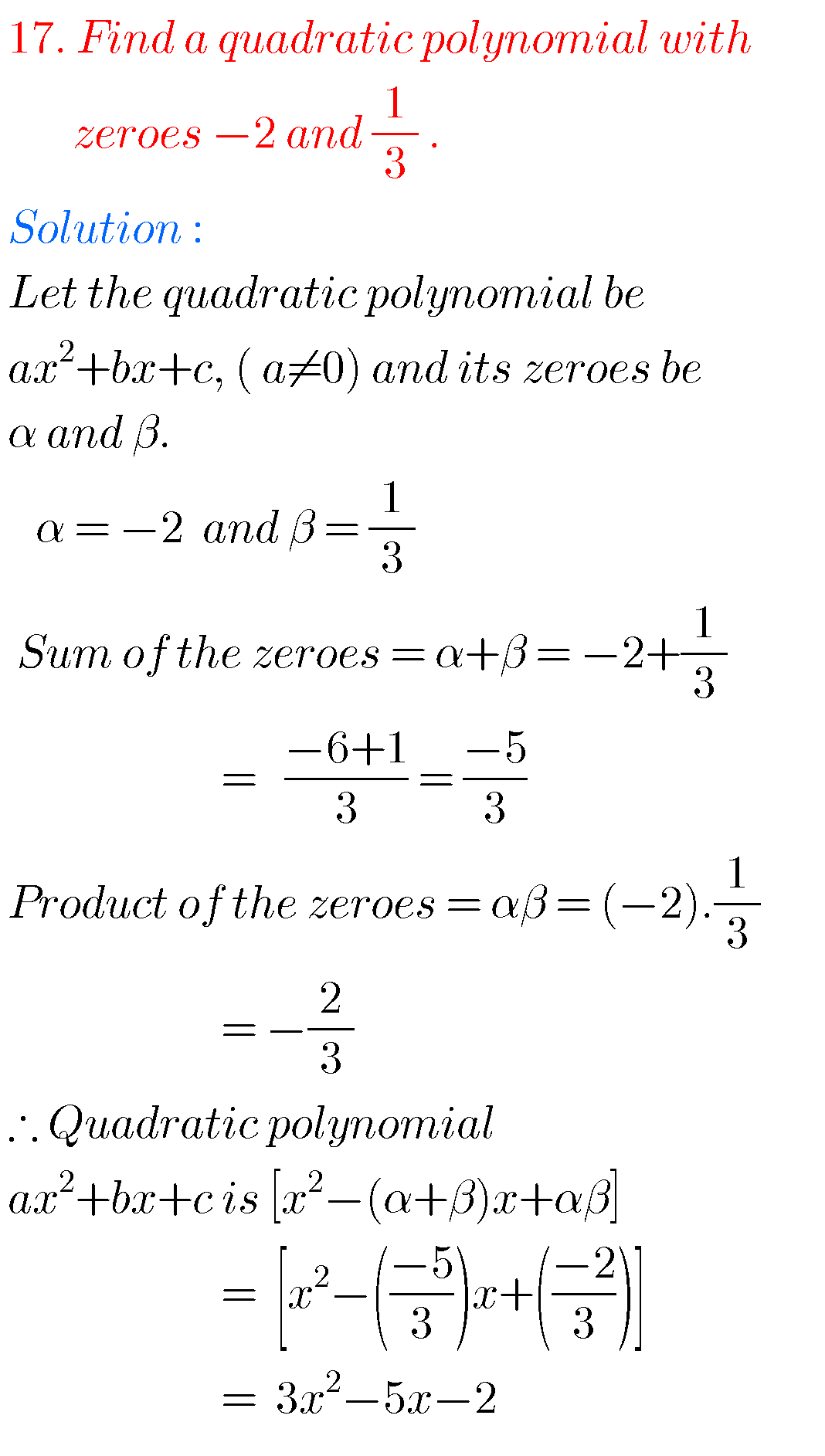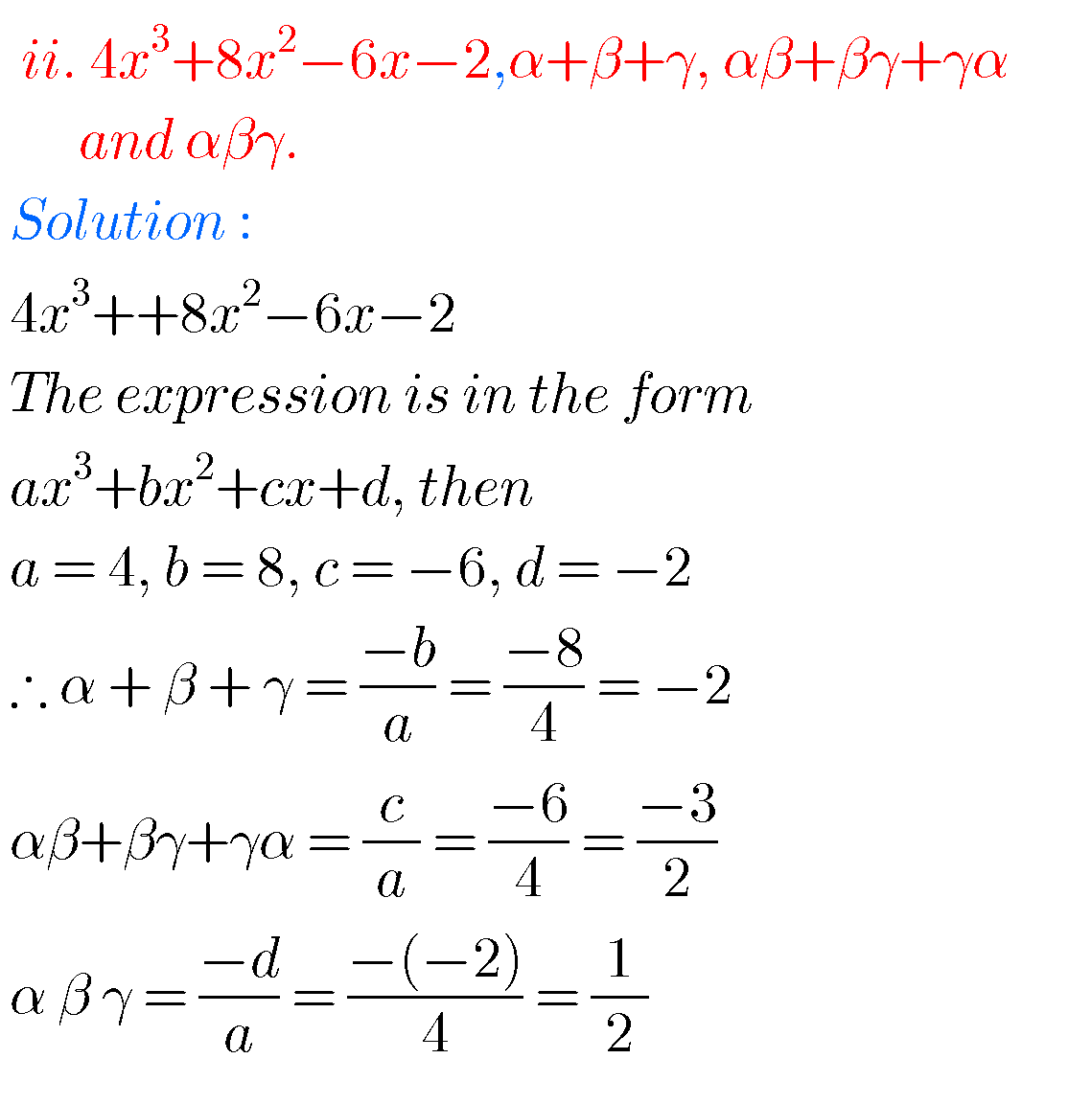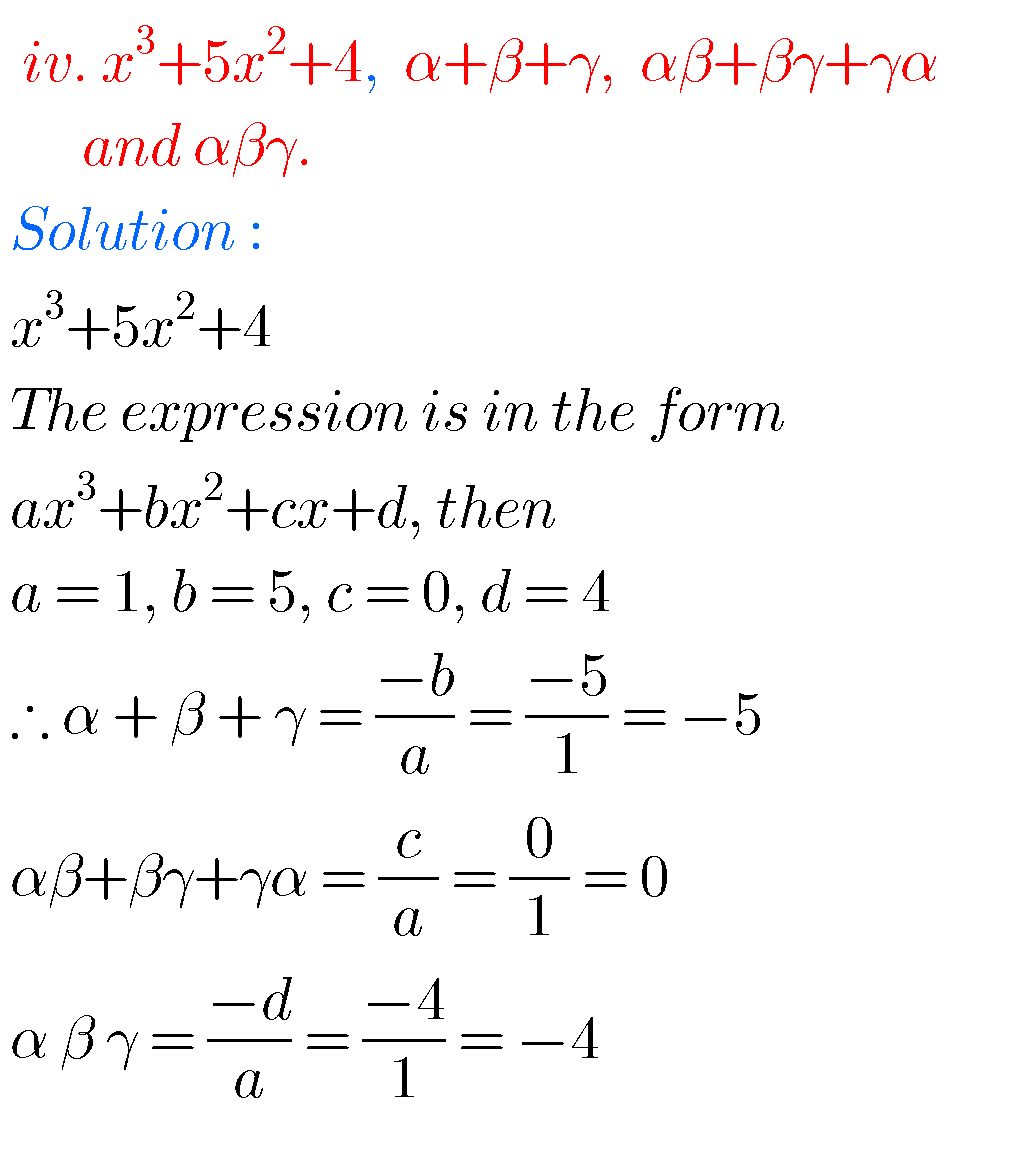Note :

Observe the solutions and try them in your own methods.

Inter maths solutions 1a

Ncert solutions for class vii factions and decimals

Ncert solutions for class viii exponents and powers